Select PageThe still small voice prepares us, “Today is Wisdom. Secrets will be revealed.”

Amongst hundreds, maybe thousands of revelations, it has never told us that before. We sense the time of this journey may be coming to an end. We may be abutting the limit of our comprehension or the limit of the Spherical Time bubble itself, the boiling conflict in Creation heating it to the bursting point.

The still small voice adds, “You will understand.”

### And G-d Said

A stiff wind blows and whistling through the 70 tall palms, it carries the whispers of the still small voice, “Vayomer Elohim (ויאמר־אלהים).”

The clear mirror promptly tells us that every time the Torah states, “And G-d said Vayomer Elohim (ויאמר־אלהים)” it is an opportunity for us to connect to the Creator and the Source, and to expand the accessibility of our consciousness. The closer to Creation, the stronger that connection, and the first time it is used is in the Torah’s 3rd verse, at the 22nd word, associating the Voice with the 22 Names and letters of the Alef-bet through which it is expressed and the Triplet Triangular form through which they are funneled into our existence.

The phrase “Vayomer Elohim(ויאמר־אלהים)” is repeated 10 times in the first 6 days of Creation and begins with “and (ו)” each time for no apparent reason.  These are the 10 Utterances of Creation. Usually whenever G-d (אלהים) said, saw, made, took, or did any action the verb is preceded with a Vav (ו) except in the Torah’s first verse because the Vav (ו) represents the 6 dimensions of Zeir Anpin through which everything we experience was created. The 10 letters in “Vayomer Elohim(ויאמר־אלהים)” represent the full 10 sefirot.

The clear mirror splits the two words into the inner 4 and outer 6 levels (ויא־מראל־הים)” and we are shown our first secret of the day, the 6 letters in G-d’s voice that correspond with the 6 bundled dimensions of Zeir Anpin equal (17 + 55) = 72, as in the 72 Triplets and the 10 letters in the spelled out YHVH (יוד־הי־ויו־הי), while the middle 4 with the kolel equal 275, representing the Primal Frequency (27.5 Hz).

We are reminded that 72/27.5 Hz= Phi(φ)2 and told that this is not a casual relationship between Phi(φ), the Primal Frequency (27.5 Hz), Creation and G-d’s Voice. You probably should not proceed unless you have read at least Chapter 32, Spherical Time. If you are ready, a whole new world awaits. The Journey begins here if you missed it.

Over the 10 times the phrase “And G-d said Vayomer Elohim (ויאמר־אלהים)” is utilized, the initials equal (7 x 10) or 70, the place of Da’at, and the 70 years at the beginning, end, and center of Spherical Time, etc., as in the 70th chapter in the Torah, when the 10 Utterances are repeated as the 10 Commandments.

They are all connected to the Source (42) through the words Vayomer Elohim (ויאמר־אלהים)” of numerical value 903, as in the sum of the integers (numerical fields) from 142, the 42nd Triangular Number.

The still clear pool nestled beneath the 70 Palms shows us that the square root of 903 is 30.04995 representing the total value of the 27 letters (4995), the mast height (300) of the Essential Triangle of Creation, the value of Elohim milui (300), and that the sum of the square roots of the integers from 1 – 30 is 112.0…, as in the 112 Triplets of Creation.

As if that were not enough, it precedes to show us that while the middle and final letters in “Vayomer Elohim (ויאמר־אלהים)” equal 336, as in the 336 letters in the 112 Triplets. Moreover, the standard value for the 2 words whose initials total 7 is 343, or 73 or 7 x 7 x 7, representing the depth of the 7 Days of Creation, and the cycles of 7, and the 7 letters that guide them through the 7 sefirot, aligned with the 7 levels of the 42-Letter Name, also represented by the same 2 words through their value (903).

Seeing everything we just learned about the 7th Day and the 72 Triplets, and just now finding out about the connection to a cube of 7, or 73, we surmise that the 9th Candle (1143343) equaling 800 must be cosmically aligned with the other 8 Candles equaling 8000.

The palm fronds smile, and the clear mirror says, “The more you know…”

And as if that were not enough, the clear mirrored pool adds that the voice of G-d “Vayomer Elohim (ויאמר־אלהים)” has the power to heal especially on the 5th and 7th Day. The katan value of the 2 words is 28, koach (כח), power, and while its ordinal value is 91, the unified Name in the center of the Path of One, its value of 343 with the kolel (2) is 345, that of the Name of healing (מהש).

### “I am G-d (אנכי־יהוה)”

It then traces the Source of the milui of the milui of Vayomer Elohim (ויאמר־אלהים)” to 15.008883 and Yah (יה).

JD interrupts the clear mirror and asks if this is connected to the 1500 that we learned about with the 2 portals linking the first Elohim (אלהים) in the Torah to the 10 Commandments.

Impressed, a breeze ripples the surface of the silvery pool and we are reminded that 107,000 letters further out from the Torah’s first word we find the words “I am G-d (אנכי־יהוה),” of numerical value 107, which has a small gematria value that sums to (1521 + 1565) = 3086, an analogue to the value of the 3rd word (אלהים), of numerical value 86.

Moreover, all 8 letters of “I am G-d (אנכי־יהוה)” knocked together equal 1500 x 105, like the final letters in “I am G-d (אנכי־יהוה)” that spell Yah (יה), 15, and like the knocked together 4 letters of both YHVH (יהוה), 1500, and the first 4 of Elohim (אלהים), 1500.

We then learn that the small gematria value of “I am (אנכי), is 1521, the sum of the 27 possible spellings of the YHVH (יהוה), indelibly tying together the 2 words “I am G-d (אנכי־יהוה)” that start the 10 Commandments and tying them to the 27 Positions of the Essential Cube of Creation.

As we scan the 27 variations of the spelled-out YHVH (יהוה) it is readily apparent that there is only one spelling for the letter Yud (י) and 3 variations for the 3 aspects each for each of the 3 letters HVH (הוה), hence 33 or 27, yet another Primal Cube.

And JD adds enthusiastically that they all connect to Yah (יה), pointing out that half of the square root of 903 is (√903)/2 = 30.04995/2 = 15.0245. The clear mirror continues being impressed and rewards us by informing us that the EB-GD gematria of the Shema’s first verse, in other words, of the 15 Triplets of the Shema, is 1521, as in the small gematria value of “I am (אנכי) and that 1521 is 392, as in the value of “YHVH Echad (יהוה־אחד), G-d is One,” which as previously taught to us is represented in the 39 letters of the 4 Name expansion of the YHVH (יהוה) that gives us the equation (232 + 39 + 4) = 275, the frequency of the Voice (27.5 Hz).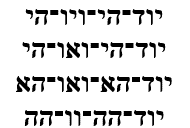We then learn that while the gematria katan of “I am G-d (אנכי־יהוה)” is 26, its complete gematria is (107 + 62) = 132, One2, and its EB-GD gematria is 151, the spelled-out value of Ehyeh (אלף־הה־יוד־הה).  We cannot help but notice that its ordinal value of 62 is also thoroughly connected to the 10 Commandments through its 62 Yuds (י) and 620 letters.

And as if that were not enough, we then learn that while the regular value of the 15 Triplets of the Shema is 1118 or (86 x 13), connecting it and Elohim (אלהים) with One and the Path of One, the value 1118 on Shabbat (702), the 7th Day, is (1118 + 702) = 1820, as in the network of 1820 YHVH (יהוה) that complete the Path of One.  While saying the Shema every day is important, saying it on Shabbat takes it to a whole new level.

The clear mirror then completes the thought process with an explanation that there are 13 letters in Elohim (אלהים) when spelled out, making 39 in all 3 aspects of it, tying it to “One.” The 1118-value of the 15 Triplets of the Shema is also equivalent to the 3 aspects of Elohim (אלהים) spelled-out plus the 4 aspects of the YHVH (יהוה).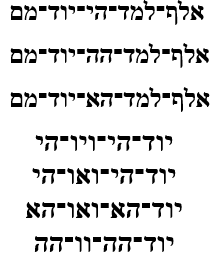The 11 letters of the Names Elohim (אלהים) and the YHVH (יהוה) combined equal (86 + 26) = 112, but the 7 aspects and (39 + 39) = 78 letters of the Names Elohim (אלהים) and the YHVH (יהוה) spelled out, turn into (300 + 295 + 291 + 232) = 1118, equaling the 15 Triplets of the Shema, which are part of the 112 Essential Triplets of Creation. This connection happens through the cycle of the 7 Days.

When that same system of gematria is applied to the 3 aspects of “I am G-d (אנכי־יהוה)” the 3 spelled-out or filled-in spelling variations add up to 1180, while the standard milui of the milui value of the 3 iterations equals (422 + 3).

Just to make sure our level of awe does not decline for even a moment, for it is awe that creates the elevation toward Creation, the still small voice tells us that the value of the 27 variations of the YHVH (יהוה) plus the 4 of Ehyeh (אהיה) and 3 of Elohim (אלהים) plus the 27 positions equals 2889, the midpoint in the Spherical Time radius and in King David’s 70-year life.

The clear mirror begins explaining that (1521 + 39) = (392 + 39) = 1560, as in 6 x the YHVH (יהוה), etc., then it fogs over and changes subject, returning to the original concept it was explaining before the welcome interruption.

### The 2 Paths of One

There are 2 words and 10 letters in Vayomer Elohim (ויאמר־אלהים)” What is this like?

It is like the River of the One that divides in 4 in Genesis 2:10; and it is like the 210 years of exile; and the 210-cubit height of the Tower of Truth, whose 20 levels match the 20 words in the 10 Utterances of Creation; the total gematria of the Torah (21009826); the sum of the 14 square roots (210.0262873…) of the 14 Triplets in the 42-Letter Name; the 210 word values in the Torah, and the Planck diameter 21.09143628… x 10-35.

The clear mirror then shows us “The One (האחד)” of numerical value (5 + 13) = 18 and the 2 YHVH (יהוה) (יהוה) from the 18 words of the 13 Attributes of G-d.  Beneath it we see the Path of One (11391455 1820) and then beneath that we see (11391455 1820) again, one path for each YHVH (יהוה). Added together, it shows us (2 – 26 – 182 – 910 – 3640) and before we can point out the obvious values of the YHVH (26) and of Jacob (182), it adds together (2 + 26) = 28 and (28 + 182) = 210. Then it adds the first 4 numbers in the series, (2 + 26 + 182 + 910) = 1120 or (10 x 112) Triplets, as in 8!/Σ8 and the 1120 cu2 of tapestries that covered the Mishkan, and it follows this up with (910 + 3640) = 4550 = (10 x 455), the 3 aspects of the Name of Binah Ehyeh (אהיה).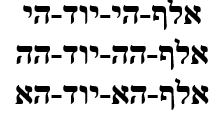The kabbalists always sing the 13 Attributes in triplicate for the 3 columns of the Tree-of-life but also to complete the 6 YHVH (יהוה) of Zeir Anpin. The 2 YHVH (יהוה) (יהוה) of the 13 Attributes connote the 2 Paths of One to Binah. Hence the 18 words and the value of 1818 of the 3 iterations of Alef (א) that form part of the 3 iterations of the expanded Name of Binah Ehyeh (אהיה).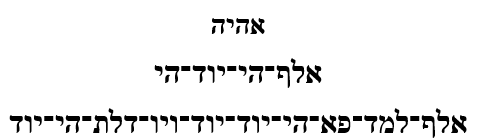The Alef (א) in that Name expands into a value of 1818; the Yud (י) in the Name expands into 506, as in the complete value of Moshiach Ben David that is associated with the first level of the 42-Letter Name of numerical value 506; and the letters Hei (ה) expand into 110, the value of miracle and the Primal Convergence point. The 3 Alef (א) expansions denote the 3 dimensions (height, width, and depth) of each of the massive 50-cubit high Gates to the Holy Temple in inches, (1375 + 275 + 165 + 3) = 1818. The 10 digits in those 3 measures of the 12 Holy Gates are (1+3+7+5 + 2+7+5 + 1+6+5) = 42, as in the final Gate of 42, the 13th Gate, and the expanded Yud (י).

Is this why “The One (האחד)” is associated with division in the Torah? Does it hint to the 2 Paths?

### Yah (יה)

The 2 words and 10 letters in “Vayomer Elohim (ויאמר־אלהים)” of numerical value 903, represents the Source, 42. What is this like?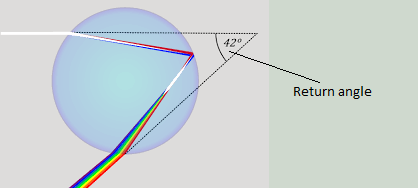It is like the final letters in “Let there be light. And there was light. (יְהִי אוֹר; וַיְהִי-אוֹר)” of numerical value 420, as in 10 x 42. Moreover, the full phrase has a complete gematria of 580 or (1000420), connecting the entire spectrum of the Light to the Source 42, just like the rainbow angle of 42o, determined by the refraction of light in water droplets.

The clear mirror adds that the phrase “On the Seventh Day (בַּיּוֹם הַשְּׁבִיעִי)” purposely has the initials (בם־הי) or 42 Yah (יה). The clear mirror now explains that the apexes of the 6 lunar ellipses around the central “earth” happen at the 15th of each lunar month and that this is because of Yah (יה), the Source of “Vayomer Elohim (ויאמר־אלהים).”

And what is this like?

“On the Seventh Day (בַּיּוֹם הַשְּׁבִיעִי)” is like Tu B’Shevat that occurs on the 15th (הי) of the lunar month of Shevat (שבט), which is 42 (בם) days after Zot Channukah and 50 (ים) days after Channukah begins, in the middle of the 150 days from Channukah to Shavuot (שְּׁבִ).  We cannot help but think that this is exactly 3 times the 50-day Omer period that hosts the final stretch from Pesach to Shavuot, which as we just learned has to do with completing the 7 x 7 cycle.

We are then informed that these are the same 150 days of the flood waters and of the 10 (י) times the 15-cubit (הי) limit of the waters above the Earth.  This is why the 6 ellipses stretch from the 15th to the 15th, the limit of Zeir Anpin.

It is all about frequency (27.5 Hz). 15 cubits, like the 15th of the month is 15 x 27.5 inches or (15 x 27.5 Hz), which equals 412.5 Hz, reminiscent of the 5 rows of the 72 Triplets that total 4120 as we just learned.

The clear mirror reminds us that the Major Seventh (M7) musical tone, as in “On the Seventh Day (בַּיּוֹם הַשְּׁבִיעִי),” is 412.5 Hz. It also advises that 412.5 Hz is 3 x the Phi(φ) angle or (3 x 137.5o), equivalent to the first letter of the Torah (בית) spelled out (412); moreover, “On the Seventh Day (בַּיּוֹם הַשְּׁבִיעִי)” is the only Day that begins with the letter (ב). This not only serves as an instruction that the first letter of the Torah (ב) = (יה) cubits and that it has a tone, but it explains why of the 7 days, the 7th is the only one to begin with an additional letter (ב), and that (7 Days x 15 x 27.5 Hz) is exactly 1.5 (יה) less than 2889, the radius of the Spherical Time equation and midpoint in King David’s 70-year life, who is associated with the 7th level of the 42-Letter Name Matrix.

### 7 Years

In the first verse of paragraph 55 of the Torah, we learn that Sarah lived 127 years as in the 7th Mersenne Prime and 127 combinations of the 7 words of the Torah’s first verse. The words utilized for 7 years, V’Sheva Shanim (וְשֶׁבַע שָׁנִים) have a gematria value of 778, yet 7 years is (7 x 355), for “Shanah שנה“ as used in the verse to describe the 20 and the 100-year periods, equals 2485, the 70th Triangular Number, and thus the sum of the integers through 70. The cycle of 7 years is as 70, the time span at the center of Spherical Time, referring to Da’at.

### There is No 3 in 72

Within the word for seven (ְשֶׁבַע) we find the permuted acronym for Ayin-Bet Shemot, the 72 Names. The clear mirror informs us that the atbash cipher for the 72 Triplets is exactly (27,000 3), reflecting the 27 positions of the Essential Cube of Creation. It further advises us that the only letter not included within the 72 Triplets is the letter Gimel (ג) of numerical value 3.  It further emphasizes this special design feature be explaining that there are 2109 letters Gimel (ג) in the Torah, as in the Planck’s Diameter 21.09143628 x 10-35 that contains the exact 9143-value of the 72 Triplets (21.09143628 x 10-35).

The palms sway and the clear silvery pool shows us the first verse of the 27 verses of the 15th Chapter and 42nd paragraph of the Book of Shemot: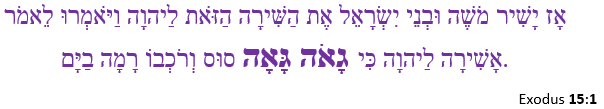Both the doubled words (גָאֹה־גָּאָה) begin with Gimel (ג) and have the value 9, but the main reason we are being shown this is that this pair is the 27th unique doubled word set in the Torah.  The root word (גָּאָה) is only found 4 times in the Torah and both times bookend the Song of the Sea. It appears again in Exodus 15:21 in Miriam’s abbreviated version of the Song.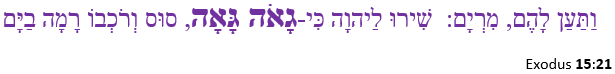The verses are then repeated to us, side by side: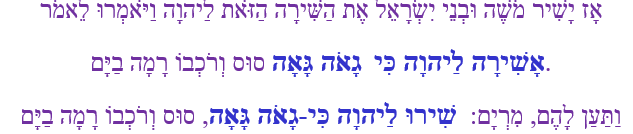The 1st verse tells us that Moses says, “I will sing to the Lord for He is highly exalted,” and in the 21st verse, Miriam says, “Sing to the Lord for He is highly exalted.”  In both cases the gematria is the same, both phrases start off with 516, as in the 516 times Moses would later pray to the Creator to bring the final redemption, and both 5-word phrases have a total gematria of 620 (keter).

The value 620 is that of the Hebrew word for 20 (esrim), as in the 20 verses between these two verses. Right in the middle of them, 10 verses from each, is the 42-Letter Sword of Moses of numerical value 6708. The 10 Commandments of 620 letters were given in Chapter 20.  This occurred 42 Days after the Endless Sea was split, and the Songs sung on the 7th Day of Pesach.  The clear mirror further advises us that of the 50 mentions of the Exodus from Egypt after the 210 years, the 7th time was on the same 7th Day of Pesach, in paragraph 42, 42 days before Shavuot.

The desert air chills, and the clear mirror takes this opportunity to explain that while the Inner Cosmos are teaching us how the Cosmic Wheels work and how our world is created and maintained, they are not astrological tools, and no one should be using the luminaries placed in the firmament, the letters that control their drive trains, or the wheels themselves as objects of divination. And while the Days work in cycles and different cyclical vibrational frequencies to which we can tune into, the Festivals are set, the Moons are set, the Shabbats are set and all Days are good days in the eyes of the Creator.

That warning established, the clear mirror returns to the two parallel verses at hand and shows us that Miriam’s Song in 15:21 is exactly 1 chapter after the splitting of the sea by the 72 Triplets in 14:21, and that the 10 verses in chapter 14 after the Sea splitting (14:22-31) plus the 20 verse surrounding the Sword equal 30 verses, as in  the Lamed (לַ) and Ci (כִּי) inserted into both phrases, both of numerical value 30, splitting this important Torah stretch into 3 sections of 10 verses each, like the 22nd Triplet (ייי).

It is then announced to us that there are 42 verses from the start of Chapter 14, as in the 14 Triplets, to the 42-Letter Sword of Moses, meaning the Sea is split and the 72 Triplets are found in the midpoint of those 42 verses.

### Crossing the Endless Sea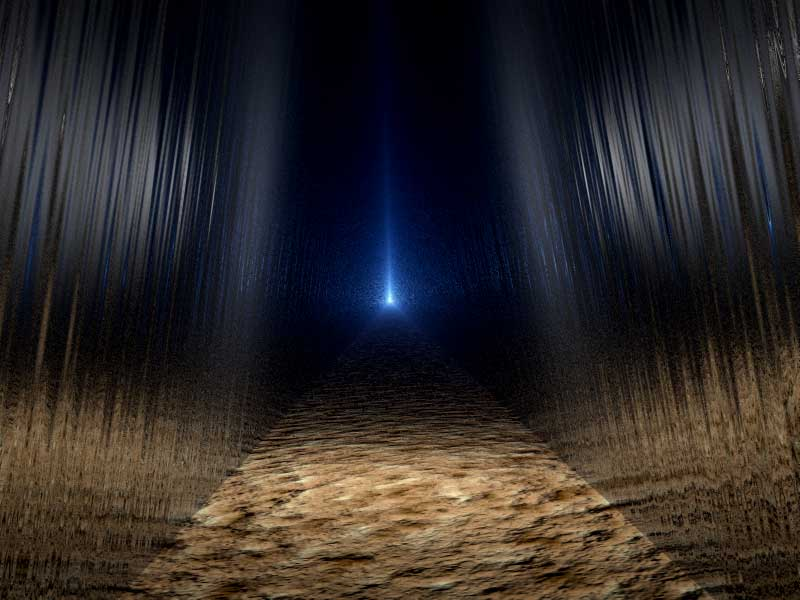The 3 verses of 72 Letters in Chapter 14 are the 19th, 20th, and 21st, as in (19 + 20 + 21) = 60, as in the hidden Samech (ס) at the exact center of the 42-Letter Name, and like the value 60 found 336 times in the Torah, as in the 336 letters in the 112 Triplets.  When those 336 times are added to the 1833 Samechs (ס) in the Torah their value becomes (336 + 1833) = 2169, as in the 9 Candles, or alternatively the 9 rows, of the 216 letters of the 72 Triplets.

A lot of planning went into the placement of the 72 Triplets and the splitting of the Endless Sea after 3 of the Israelites 42 journeys, along with the concealed tool of the Sword of Moses. It dawns on us that maybe there is a concealed message in there as well. Considering, 99.9% of Man’s technology appears to have appeared only in the 19th, 20th and 21st centuries perhaps this message about enslavement and freedom after 210 years in verse 21 is about us in the 21st century. Perhaps all the messages about 21.0 and 2100 and 210 are about us?

The 210 years come to an end in the 21st century.

The Splitting and Crossing of the Endless Sea is the 4th Journey of 42 journeys of the Israelites. There were 3 journeys that proceeded it and that in verse 14:2 stopped at “Pi HaChirot (החירת פי), Freedom Valley, between Tower (מגדל) and the Sea.“

Could the Tower be the 210-cubit and 210-level tall Tower of Truth, with its encasing Pyramid?

After all, the atbash of “Tower, Migdal (מגדל)” conceals a value of 330, as in the 330-cubit base measure of the encasing pyramid. Moreover, “Tower, Migdal (מגדל)” has a numerical value of 77, whose square root is 8.775 or 5778 in reverse, and while this was the 3rd Journey described in verse 33:7 of the Book of Numbers we know that the 11 Triplets of Creation is comprised of 33 letters that equal 3003, the 77th Triangular Number. We also know that this occurred in 2448 HC, 3330 years before 5778.

Regarding “Pi HaChirot (החירת פי), Freedom Valley,” of complete numerical value 805, as in the rainbow, the sign and covenant that the Creator made with the Earth after the flood, we know that rainbows are made possible only because of the 42o refraction angle of the light traveling through the raindrops.

The Israelites made 3 journeys while still in limbo in Egypt. Thanks to an act of G-d, during the 4th journey they crossed the Endless Sea, and into freedom from the 5th on. What is this like?

It is like the commandment that “…for 3 years [the fruit of the newly planted tree] shall be a forbidden growth, and may not be eaten. Then, in the 4th year all [the tree’s] fruit shall be holy, and it shall be something for which God is praised. In the 5th year, you may eat its fruit and thus increase your crops. I am God your Lord.”  These 3 verses in Leviticus chapter 19: are verses 23, 24 and 25, which total 72.

The earth rumbles and the silver liquid crests upward in two directions, forming a giant trough, as the clear mirrored pool splits down the middle for us. With tall silvery walls on either side we enter with trepidation. On one wall we see the 42-Letter Name Matrix. Opposite it we see the Name (החירת פי) with 3 letters aglow: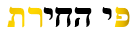The same 3 letters then begin to glow within the Matrix.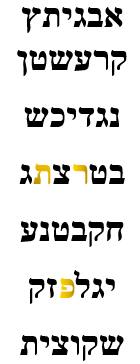We then see that they form a triangle.  Briefly another triangle is formed overlapping the Pi-Charoth Triangle. Its numerical value is 72.  The letters (צט) trapped in the middle equal 99 and (9972) = 27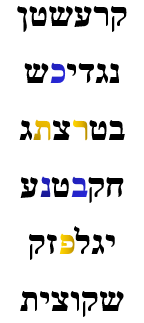It vanishes, but 4 other letters glow in its place, and we instantly realize that the Pi-Charoth Triangle represents the last 3 of the 7 letters of the 7 Planets. Those 4 letters with the kolel have a numerical value of 33, as in the verse of the 3rd journey, verse 33:7 where we find the Israelites camping between the Tower and Freedom Valley.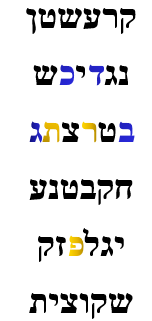We are advised to remember the Triplet (פרת) and its triangular shape as the silver walls begin to reverberate and start to bulge toward us. Before they destabilize and come crashing back, we rush through the dry sandy passage and dive onto the desert shore.

The still small voice asks, “What, no song?”

There is no Gimel (ג) in the 72 Triplets because it was established in the 3 verses of 72 Letters that split the Sea, and it is in the middle of The Garden (הגן) of numerical value 708, the value of the concealed 42-Letters of the Name YHVH (יהוה).

The Song of the Sea is supposed to be a prophetic song about the coming of Moshiach and after Moses says (alludes to) that he will sing/pray for the final redemption and Miriam tells us to sing/pray for it, the unique double words highly exalted (גָאֹה־גָּאָה) appear of numerical value 18, as in the square of Moshiach Ben David, Chai, and “The One.”

Speaking of “The One (האחד)” another set of doubled words is also associated with it.  The doubled Name Abraham Abraham is the 13th unique one and 18th overall.

The clear mirror uses the gematria of Abraham of numerical value 248 to segue into the 248 dimensions of the Torah and the E8 Lattice, but this time it shows us the Essential Cube of Creation with its 27 positions and parallel Magen Davids.

### A Tale of 10 Sefirot

But first, it wants to make one more connection for us to the 72 Triplets and it begins by telling us that the doubled word (גָאֹה־גָּאָה) value of 18 is connected to the double Tets (טט) in Metatron (מטטרון) of numerical value 314, Pi (π). It next explains that the first 3 letters in the 72 Names, its first Triplet (והו), has the numerical value of Tov (טוב), good, that begins with the letter Tet (ט). When added to the next Triplet (ילי), the first 6 letters equal 67, as in Binah, making the first 7 letters equal to 127, as in the 7th Mersenne Prime, Sarah’s age, and the combinations of the first 7 words. While the 8 first letters of the 72 Triplets equal 137, kabbalah, meaning to receive and parallel, the 3rd letter of the 3rd Triplet, the 9th letter, is Tet (ט). This gives the first 3 Triplets the value 146, as in Olam (עולם), world/universe, as in the 4th Triplet (עלם).

The clear mirror reminds us of the equation 9/.1230490273… = 73.14157771495…, alluding to the Cosmic Harmonic 273 built into the 42-Letter Name, the year 5777 HC, and the sum of the 22 Letters of the Alef-bet, 1495, plus 70 and Pi (π). It continues showing us that 73.14157771495…/2 = 36.5708 or the two sets of (גָאֹה־גָּאָה) of numerical value 36, which are the 35th and 36th overall doubled words in the Torah. It is of course most notably the 365 Solar Days and the date 70 years earlier than 5778, the year 5708 HC, when Israel became a nation. And this includes of course the 708 value of the Upper 42-Letters of the Name YHVH (יהוה) that are so integral to the Cosmic Wheel and that we just saw with “The Garden (הגן).”

Ten digits 1 – 9 are associated with Hebrew letters, and letters have multiple values depending on which cipher is applied. The complete gematria cipher of the letter Tet (ט) that is associated with the number 9 is 18. The clear mirror shows us this just to remind us that when the value 18 replaces 9 in the above equation (18/.1230490273…) the result is 140 (עלם), the 4th Triplet, plus 2π, or 146.28…, the numerical value of Olam, (עולם) the world/universe and the value of the first 3 Triplets of the 72 Names.

It then casually throws out there that the albam gematria cipher of those first 9 letters/digits totals 720, as in the 9 Candles of the 72 Names.

Almost reluctant to move on, the clear mirror explains these connections all take place in the 9th Candle, which is mostly associated with Chanukah and Binah. It waits for us to picture the 8 Triplets in the 9th Candle and tells us that if we want to learn like a Kabbalist, we must learn to bring the Names into our hearts and see them with our souls. The Torah is constructed with 3 Upper sefirot (dimensions) and 7 relatively lower ones of Zeir Anpin. The Torah is constructed with 2109 Letters Gimel (ג) of numerical value 3 and 2198 letters Zayin (ז) of numerical value 7. The difference between them is 89, as in Chanukah (89) and the 89 Days from the Day of Creation to Chanukah. Together with the YHVH (יהוה) of value 26 they represent not just the complete 10 sefirot but the 21009826 total gematria of the Torah. Together (2198 + 2109) = (4240 + 67), Moshiach Ben David and Binah (67), the value of the first 2 Triplets of the 72 Names. It concludes by telling us that while the Name Zayin (זין) of numerical value 67 represents Binah, the 8th Sefirot and the Name Gimel (גמל) of numerical value 73 represents Chochma, the 9th Sefirot, the 2 Names Gimel (גמל) and Zayin (זין) together equal (73 + 67) = 140, the 4th Triplet of the (8 x 9) Matrix, as was just taught to us.

If we think of the letters as Angels of G-d, each with their own personalities, backgrounds, and unique ways of interacting perhaps then we would treat them with more respect and awe, and realize the masterly way that hundreds of thousands of them work tirelessly in concert.

The clear mirror decides it is time to continue its discussion of the Magen Davids in the design of the Essential Cube of Creation. It reminds us that at the instant the concept of numeration was conceived it was also determined which numbers would be prime numbers and thus that the value 378 would also be the sum of the digits in all the prime numbers through the 42nd Prime, the number 181.

I guess it wanted one more parting shot at the 72 Names before actually moving on, because it explains that 181 is 35 more than the numerical value (146) of Olam, (עולם) the world/universe and sum of the first 3 Triplets of the 72 Names, and 35 less than 216, as in the 216 letters in the 72 Names (Triplets), just like the 35-year split in King David’s 70 year life at the exact center of the Spherical Time radius in 2889 HC.

### The Magical Magen David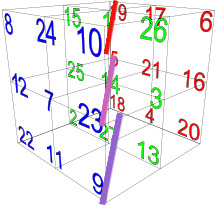There is a sweet and subtly spicy smell wafting through the palms. It is at once enticing and soothing, a hint of vanilla, cinnamon, frankincense, hyssop, and cloves but more complex. We cannot see its source but it reinforces our sense of being on an island oasis, surrounded by desert dunes.

Our attention is drawn back to thecenter of the oasis, dividing the 27 positions/letters of the Essential Cube of Creation into 9 groups of 3 brings up the gematria cipher AYK-BeCaR.

Aha! Now we know why the clear mirror began with the discussion about the Gimel (ג) and Tet (ט), 3 and 9.

As previously explained, this cipher groups the letters of similar value and exchanges their places (1-10-100, 2-20-200…9-90-900). For the purpose of forming Triplets, we get (איק), (בכר)…(טצץ) or (111,222,333…999). Nine (9) Triplets in the Essential Cube of Creation, along with the other 112, make 121 or 112 in total, as in the 11th sefira, Da’at, and the connection to the complete value of Moshiach Ben David through the value of the 42-Letter Name’s first level, 506, which equals the sum of the square from 12 to 112.

The middle Triplet (הנך) of the 9 has the value 555, and is known to represent the Shechinah, or the feminine, or earthly (Malchut) aspect of the Creator, in the same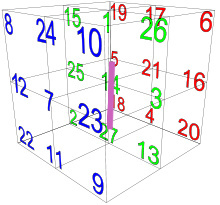way that its composite letters and their associated numbers, 5, 50 and 500 represent the feminine aspect. We have also previously explained this concept of the relationship between feminine and male in the more cosmic terms of squaring the circle and as receptive and projective duality energy forces/pressures.

When converted to their ordinal values these 3 letters (הנך) correspond to the numbers 5 – 14 – 23 respectively, as in the bar or vector that diagonally pierces the middle of the cube. This necessarily means that the sum of this pivotal vector is 42, and mathematically this is the only one of the above 9 triplets that can be 42, thus associating the Shechinah with the number 42.

In case we were to think this alignment of the Shechinah letters is nothing more than surreptitious, the Creator placed the final of these 9 Triplets just below it, the 9 – 27 – 18 Triplet or (טצץ), 999. And right above it is the 10 – 1 – 19 or (איק), 111 Triplet.  This design element was obviously crucial in the cube’s functionality, not because of mathematics but because it is the Cube of Creation, the cradle of Creation.  Everything in the Torah and Creation was channeled through is 7 dimensions.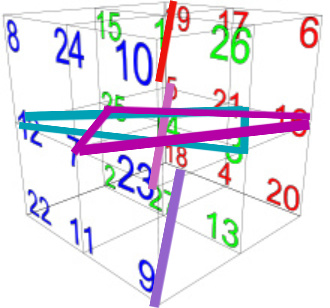Moreover, the 2 – 11 – 20, or (בכר), 222 Triplet is found as a triangle in the bottommost horizontal plane, as is the 4 – 13 – 22 or (דמת), 444 Triplet; the 3 – 12 – 21 or (גלש), 333 Triplet is found as a triangle in the middle horizontal plane, as is the 7 – 16 – 25 or (זען), 777 Triplet; and the 6 – 15 – 24 or (סםו), 666 Triplet is found as a triangle in the uppermost horizontal plane, as is the 8 – 17 – 26 or (חפף), 888 Triplet.  It is a little hard to tell from the perspective in the graphic, but the triangles on each plane intersect to form Magen Davids (Stars of David) with the cross bars going horizontally right through the centers of each one. This is rather astonishing.  If we look straight down through the top of the cube, we would see a single strong Magen David (Shield of David) with a bar across the middle. This is the true origin of the Magen David, created at the origin of time, not halfway through our civilization.  And in the very center of that Star is the number 14 for David (דוד).

Furthermore, the average of each star is (222 + 444)/2 = 333; (333 + 777)/2 = 555; and (666 + 888), = 777, mimicking the triangles and bars of the middle plane 333, 555, 777, while the average of the 3 parallel bars is (111 + 555 + 999)/3 = 555, once again giving us the Shechinah in the center bar of the center plane.  The Magen David is the symbol of Israel, and the 555 value of the central bar and/or plane minus 14, the central position = 541, Israel—conceived at the dawn of time.

In terms of the numbers, the 3 Stars and 3 Bars have to sum to 378, the value of “Echad Ushmo Echad (אחד־ושמו־אחד)” or “His Name is One,” whose final letters spell David (דיד), because they represent all 27 ordinal values. Nonetheless, the ordinal values of the uppermost Star do not have to equal 72, but they do. The middle plane Star and Bar do not have to equal 126 or (3 x 42) with the Star being equal to 84 or (2 x 42), but it does. And the top and bottom plane Stars and Bars do not have to together equal 252, or (6 x 42), but it does as well.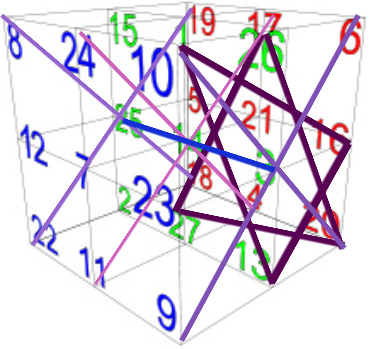There are, though, 3 axes to the cube, and as it turns out the 3rd axis also forms 3 parallel Stars of David with crosshairs that also sum to 6660. And just like the other two axes there is a ratio of 15 – 150 – 1500 built into its 3 tiers or planes.  Just like the second axis, one of the triangles in the front vertical plane (20,23,26 רךף) sums to 1500; a bar (11,14,17 כנפ) in the middle plane sums to 150; and a triangle (2,5,8 בהח) in the rear plane sums to 15.  The 3rd set and axis is perpendicular to the other 2 sets and axes.

While this progression of Yah (יה) from 1 to 10 to 100, or 3 orders of magnitude, in each of the 3 tiers in each of the 3 axes is of paramount importance in the Cube’s Divine design, the 3 sums of (15 + 150 + 1500) x 3 total 4995, the sum of the 27 Hebrew letters.

The astonishing part is that the 27 letters used to compile these 3 sets that sum to 4995 are not the same Hebrew letters from 1 – 27 that also sum to 4995 because some are duplicated, and some are not included at all.

When we go from 1 to 10 to 100, we are expanding from Malchut to Zeir Anpin to Binah.  One of the Arizal’s Rosh Hashanah kavannot is to draw down the 150 Lights, specifically as 10 x Yah (יה), as specifically spelled out (עשר) in the 2nd level of the 42-Letter Name.  The first and last letters (קן) in this line/level also equal 150.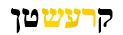If you recall, the vertical axis Stars were constructed entirely out of similar letter values, like 222 or 888.  When we examine the counter rotated or opposite Stars in that same vertical axis, all 3 of them are wholly comprised of triangles that sum to either 15, 150, or 1500.

The uppermost plane on that axis has a triangle of 150, and another of 1500, and a bar of 15, and with the other bar of 111, it gives us 1776. The middle tier one has a triangle of 15, and another one of 1500, for a total of 1515. The bottommost tier has a triangle of 15, and another of 150, with a bar of 1500.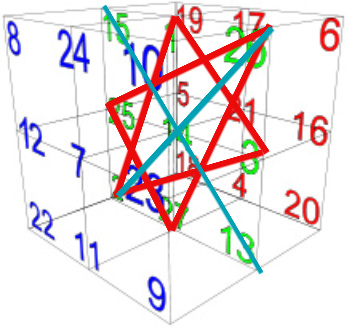Another date baked into the Magical Cube of 42 is found in the sum of the Star of David (2-1-3; 26-25-27) with its crosshairs (13-14-15; 2-14-26) in the middle of the 2nd plane of the 2nd axis, which is 3408, as in the year 3408 HC, the year the 2nd Holy Temple was built.

It is also interesting that the sum of the crosshairs of the rear or 3rd plane of the 3rd axis is 1998 or (3 x 666), as in the first 5 words of the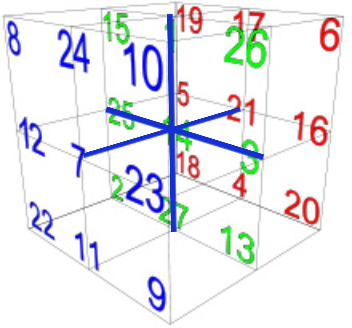Torah that total 1998.

Remember, this is a cube and as such it can be rotated along any of the 3-axes, so none are more than momentarily vertical or horizontal, and no face is any more considered the rear face than it is the front one.  All 3 sets of Stars of David with their crosshairs equal collectively (3 x 6660) or 19980, which also equals (4 x 4995), the sum total of the 27 letters in the Bird’s Nest. And in case we did not get the point in the previous sections, 4995 = (45 x 111) and (3 x 6660) equals 19980 = (18 x 111 x 10) and the square root of 18 is 4.2426….

The Essential Cube of Creation fades away, leaving only the Magen David, which begins to grow and rise above the still cool smooth pool, expanding ever upward. In each of the 6 vertices of the Magen David we see a separate Cube of Creation, 27 positions in each vertex, as if each of the 6 faces/dimensions of the original cube became its own Cube. The clear mirror advises us that there are 27 dimensions in each vertex of this Magic Star. In the center of the Star, where the 14th position of David began, there are now 78 dimensions, as in the 3 YHVH (יהוה).  The complete value (64) of the letter Nun (נ) that was there is now added to the 14th position to become 78.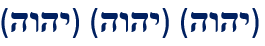The clear mirrored pool below adds that the 78 dimensions align with the 78 letters of the Names Elohim (אלהים) and the YHVH (יהוה) spelled out.As the massive Magic Star keeps expanding, we see that there are 6 vertices further out, surrounding the Star. Each 27th dimensional vertex is associated with a 1-dimension outer vertex, 28 in total, like the letters in the Torah’s first verse, 2701. A little back of the envelope arithmetic shows us that there are (28 x 6) dimensions or (4 x 42) dimensions surrounding the central 78, making 246 in total.

The clear mirror now reveals that we are observing a 2-dimensional projection of the 248 dimensions of the E8 symmetry, 246 plus the 2 in the projection. The 27 in each of the star’s vertices represents the 27 vectors off each of the 6 outer vertices. The 6 outer vertices exist in the E6 symmetry sliced from the E8.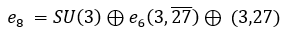The actual equation looks something like this, which means nothing to us as the Magic Star vanishes and the clear mirror returns to the Torah, the true projection of the 248 dimensions that keep unfolding for us fractally moment by moment.

### The Nature of 3

Referring to the 3rd order of magnitude of Yah (יה) that we saw repeatedly in the Essential Cube of Creation, 1500, the clear mirror shows us that while 3 is the lower limit and 1500 is the upper limit in the gematria of any of the 210 word values in the Torah matrix.  Within those 8(10,0003) words within the Torah’s (248 x 42) matrix exactly 68 of them have the value 3, as in Chaim, life.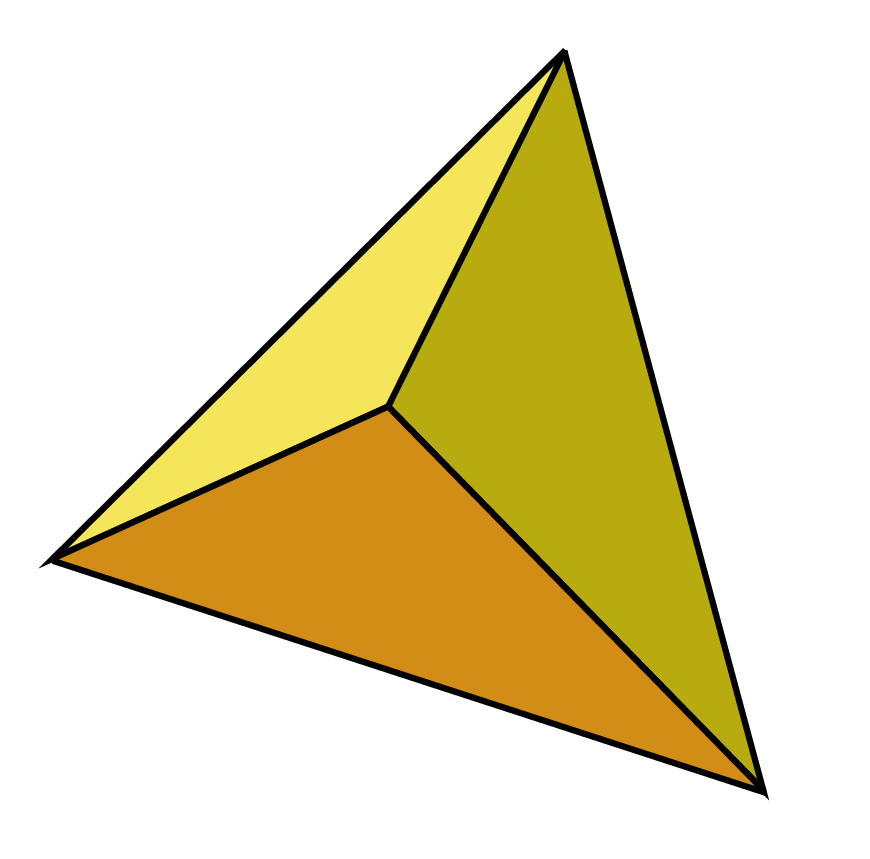Just when we think the Inner Cosmos is going to the tie in the triple sequencing of the codons of our DNA, it reminds us instead of the triangular cartouches we saw in the branching tunnels at the start of our journey, just after we left the 248-word cavern of the Shema.  Like the 401,273 elements of the Torah that equal 248,000φ there are numerous relationships within the quasicrystals of the 248 dimensions of the E8 symmetry, all the way down to the 3-Simplex, the basic 3-d symmetrical unit, the Tetrahedron.

The clear mirror explains that the Torah Triplets that we have been previously shown as 2-d triangles representing 3 loci in hyperspace, 3 address in the upper dimensions, are actually Tetrahedra, the first of the 5 Platonic solids. There are still only 3 loci represented by the 3 letters but the 4th vertex in the tetrahedron is the Triplet, the combination of all 3. This holds true for the 4 faces of the tetrahedron as well, 3 letters and one combined Triplet. The Triplet is on the face opposite the vertex with the Triplet

It is then explained that the 6 edges of the tetrahedron unfold into the 6 dimensions of Zeir Anpin that form the 6 directions/faces of the Essential Cube. As we picture this unfolding, the clear mirror advises us that like the 6 faces, 12 edges and 8 vertices of the Cube that equal 26, the YHVH (יהוה), the Tetrahedron’s 4 faces, 6 edges and 4 vertices equal 14, the central position of that Essential Cube.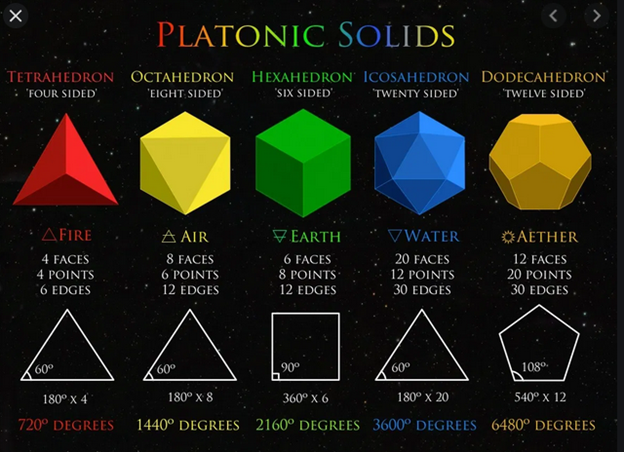The unfolding was a little complicated for our simple processing minds, so the clear mirrored pool obliges us and show us that each 2-d edge of the tetrahedron becomes a hyper vector and expands into a 3-d tetrahedron of its own and the 6 resultant Tetrahedra fit together like perfect pieces of a puzzle into a cube. Pretty cool!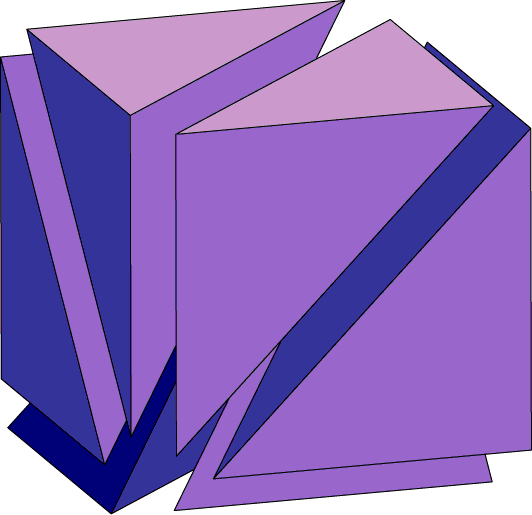This is not surprising as the Tetrahedra are the smallest pixels of the 3-d picture we call reality.

It is also not surprising that the triangular facial angles are 60o as the value 60 is found 336 times in the Torah, as in the 3 x 112 letters in the 112 Torah Triplets, and as 60 is the value of the hidden letter Samech (ס) in the center of the 42-Letter Name.

So doing a little arithmetic of our own we calculate that with the 3 letter Triplets expanding 6-fold, there are 18 letters for each of the 27 positions in the Essential Cube of Creation, which we know is a magic cube of 42. We see Napier’s Constant (e), 2.718… and are shown that (27 x 18) = 486, which the clear mirror reminds us, is a word value (486) that is found 42 times in the Torah.

Moreover, 486 is not only part of the 291st Triangular Number, as in the last 3 letters of the Torah’s first verse (ארץ), earth, of numerical value 291, that is 42486, but 486 is the sum of the first 6 age differentials in the first 7 generations of Adam, through Enoch, who walked with G-d at age 365.

Every time the Inner Cosmos displayed the Tower of the Generations we learned more and went deeper. The Torah is an endless matrix of fractal crystals. As an exclamation point, the clear mirror adds that 4 values are found 42 times (756, 547, 703 and 486) in the Torah. We have seen all of them in our journey. Together, they sum to (756 + 547 + 703 + 486 4) = 2488, the Year Moses, the 26th Generation, dies and the Israelites enter Israel.

And as for the 291st Triangular Number, 42486, we can see immediately the sum of the 22 Names of the 22 Letters, 4248, and 424, Moshiach Ben David with 86, Elohim.

That was for fun; this is for Understanding. When we question which of the Triplets apply to the 27 positions of the Essential Cube of Creation that got expanded into 27 x 18, the clear mirror first corrects us. The expansion was just the perspective from a higher dimensionality; the Essential Cube as we know it is the projection into 3-d, the limit of our processing power.  It then explains that the 27 Letters associated with the 27 positions of the Cube are all representations of their 3-letter Names. Before we can object it explains that of the 22 Letters whose Names total 4248, there are 17 Names of 3 normal letters, and 5 that contain 2 each plus 1 null or counterspatial letter, for 66 in total. These 5 are akin to the 5 final letters that exist yet do not exist independently. Their spatial position is not in a void; it just does not have a physical value. At 66 letters the 4248 average is 64.363 per letter, as in the central value of the Essential Cube (14 + 50) and as in the 64 codons and the 64 or 43 null counterspatial combinations of Torah’s first 7-word verse.

The geeks in our group immediately calculate that (4248 + 66) = 4314, bringing in Pi (π), once again and Metatron (מטטרון). Funny how that works in the Inner Cosmos—almost as if the Creator has a plan.

### Back to the 7th Day and Hyperspace Mathematics

The discussion of the 7 words of the first verse that are analogous to the 7 Days of Creation and the relationship with Pi (π)—also built into that verse—lead the clear mirror to bring up the product of the letters in the word 7th (הַשְּׁבִיעִי), which is (5 x 300 x 2 x 10 x 70 x 10) = 21000000, as in 42 x 500,000. We are reminded that 500 parsang is the distance between Zeir Anpin and Binah, Heaven and Earth, and that the total gematria of the Torah divided by the 5-digit repeated sequence that caps the first 1000 digits of Pi (π), 21009826/42019 = 500.007758395

With the product 21000000 of the 7th Day just 9826 less than the 21009826, the total gematria of the Torah, the clear mirror reminds us that the P/S ratio of 21009826 is (2 x 1 x 9 x 8 x 2 x 6)/(2+1+0+0+9+8+2+6) = 1728/28 = 123/28, whose 4th root is 2.802828, as in the 28 letters of the Torah’s first verse, and the square root of the number of words in the Torah, 282.8002828. And circling right back to the 7th Day, this is like the sum of the 7 cubes for all 7 Days, 784, or 282, power (כח) squared.

Kicking into high gear, the clear mirror continues explaining that the product of the letters (2 x 10 x 6 x 40) in B’yom (בַּיּוֹם) is 4800, as in the 304805 letters in the Torah, and thus the two words “On the Seventh Day (בַּיּוֹם הַשְּׁבִיעִי)” total 21004800, which is 5026 less than 21009826. It then reminds us that the 9826 component is found in the middle of the mysterious repetitive 18-digit sequence (173553719826446289) from the Phi(φ)-generated Fibonacci Numbers, and that the sum of the 14 square roots (210.0262873…) of the 14 Triplets in the 42-Letter Name is off from 21009826, the total gematria of the Torah, by (2100982621002628) = 7198, as in the …7198 digit string that overlaps 9826 in .173553719826446289.

What is odder yet is what the sum of the 14 square roots (210.0262873…) of the 14 Triplets of the 42-Letter Name have in common with the 72 Triplets through the Planck Diameter (21.09143628). Beside the obvious 210 and 628 that are in both irrational numbers—one the fundamental distance in physics, and the other the fundamental source in hyper physics and spirituality—the 2628 in the sum of the 14 square roots (210.0262873…) of the 14 Triplets is equivalent to the sum of the integers from 1 – 72, the 72nd Triangular Number, while the 9143 in the same position within the Planck Diameter (21.09143628 x 10-35) is the sum of the 72 Triplets.

Also, the next number, the 73 in 210.0262873…, is the Triangular Number representing the value of the Torah’s first verse, but before segueing into the 72 Names, the clear mirror informs us that another constant of physics related to the Planck diameter is in Heisenberg’s Indeterminacy Equation.  The uncertainty of position times the uncertainty in momentum is greater than or equal to h/4π, or 5.272859 x 1035 Joules per second, with 2728 being the sum of the two common components (2100 + 628).

Like the 9 Candles of the 72 Names, the sum of the 18 final letters in the 9 Utterances of Creation “Vayomer Elohim (ויאמר־אלהים), is 7200, as in the sum of the square roots of the 72 Triplets of Creation, 720.0….

What this means is that the sum of the 14 square roots (210.02628…) of the 14 Triplets in the 42-Letter Name plus the sum of the square roots of the 72 Triplets of the 72 Names, 720.0…. equals (21002628 + 72002) = (21009826), the total gematria of the Torah (21009826).

How mind blowing is that! How on earth did the Creator do that?

By now, you would think we would be completely jaded.

### The Mysterious 18-Digit Sequence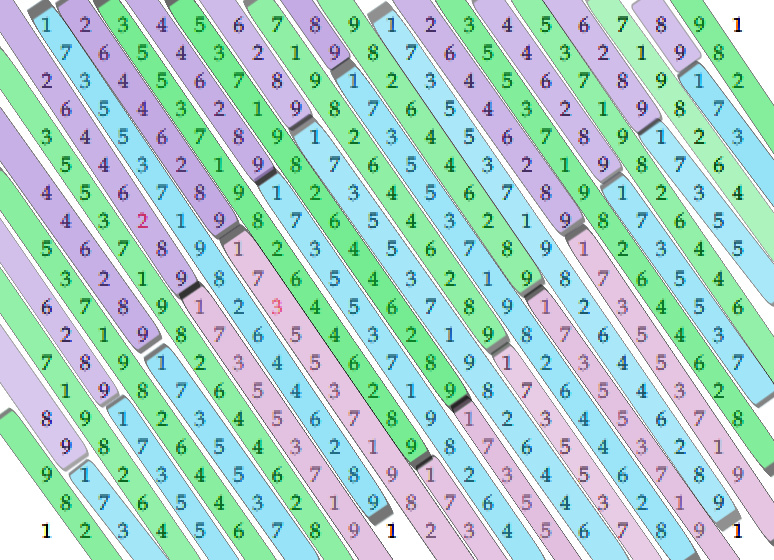That mysterious 18-digit sequence keeps popping up in the strangest places, and as we recall it was also derivable from the volume of the 21st level of the Tower of Truth. It is a key code of some sort.  The mystery deepens as the clear mirror explains that when the sequence (173553719826446289) is split up into summable even components like 18 single digits that add up to 90, and 9 double digits, 6 triplets, three 6-digit sequences, and two 9-digit sequences, the cube root of the 38 separate components is 1000.447555. This immediately reminds us of the sum of the 72 square roots of the 72 Triplets and the 9 square roots of the sum of the 9 rows of those triplets that equals 1000.054, and also of the first 1000 digits in Pi(π) that sum to 4470 that we find in the circumference of the Spherical Time equation 2(5778π) = 2π(3760 + 2018) = 36304.24470. This seems all the more appropriate given that that 38-component sum is (9 x 111260363).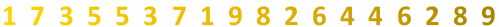We are then shown the 18 digits engraved into the dark sky. Beneath each digit is its square root, which are then summed up for us. It turns out that their total is 38.61200 as in the 38 separate components and the numerical value of the word brit (ברית), meaning covenant.

Those square roots vanish and are replaced by the 18 fourth (4th) roots, which total 26.040… We are unmoved until they are replaced by the 18 logarithms, whose total is 11.119526… or 11.12 as in the 38-component sum (9 x 111260363).

Finally, we are shown the natural logs based on Napier’s Constant (e) and these 18 values sum to 25.60365496. Our backs stiffen as we recall that 1/25.60 = 390625, the exact number of words, verses, and letters in the Torah. We also know that there are 365 prohibitive mitzvot in the Torah tied to the 365 solar days and that 496 is twice the 248 positive mitzvot and the numerical value of the 7th level of physicality, the 10th sefira, Malchut when counted from highest to lowest.

Just for the heck of it, we ask the clear mirror what 173553719826446289/5778 is and it promptly replied 173553719826446289/5778.28 = 3.00355330352. We know at once this is a purposeful connection of the year 5778.28 to 3 and the implied but hidden Gimel (ג); to the 3003 total of the 11 Triplets of Bereshit; and to the value of the Hebrew word for year, Shanah 355; to the frequency of the Perfect Fifth (P5) or 330 Hz, and the (12 x 27.5) time quotient and cubic perimeter.   We also know there must be more to this cosmic puzzle.  Maybe that is why 173553719826446289/Phi(φ) = 10726210, after all 5778 is the 107th Triangular Number and Phi18 = 5778.

What we did not recall so quickly is that at the 173rd digit in Pi(π) is the string …2110555… which is the number of days in 5778.28 sidereal years with 173 being the small gematria1, of the 42-Letter Name, or that …2701…, as in the value of the Torah’s first verse, is found at digit # 165, the small gematria0 of that same 42-Letter Name Matrix.

The clear mirror also wants us to know that the digit sequence …300355.. of the resultant 3.00355330352 is found in Pi(π) at digit #1358, as in the numerical value of the Baruch Shem that should be recited silently after each of the 5 Essential Elements of Creation, to help engage our minds to them and to Binah, the Consciousness level, and to 358, Moshiach Consciousness.

### One, Not the Loneliest Number

We try to process this, yet we are not given pause as the clear mirror continues, reminding us of what we had previously learned from R’ Azulai of blessed memory in L’Chesed Abraham that the value of Alef (א) should be taken as 1818, its value after 3 iterations, making the two of them intrinsically included in the Bet (ב) of B’yom (בַּיּוֹם) and of Bereshit equal to (2 x 1818) = 3636 and that 21009826/3636 = 5778.28, while the 6 levels in the 2 Alefs (אלף) can be interpreted as (6000222) = 5778, the Spherical Time radius, and event horizon.

We know that Echad (אחד), meaning One has a numerical value of 13, as in the 13 Candles that comprise the 112 Triplets of Creation. What we did not understand until now is the relationship between Echad (אחד), meaning One, and Moshiach (358). It has to do with the Primal Frequency (27.5 Hz), in that 358/27.5 Hz = 13.01818.

Referring to frequencies again, it is explained to us that the sofit value of Alef (אלף) is 831 and since the apexes of the lunar ellipses occur on the 15th of the month or lunar cycle, the frequency at the 2 apexes, the 2 further out points, of each of the 6 lunar ellipses is (15 x 27.5) x 2 = 825 = (8316).

### It is the Frequency

Moreover, we are reminded of the equation of the 20 cubes of the Tower of Truth, 20 x 27.5(1 1/φ) = 210.0 that expresses the relationship between Phi (φ), the Primal Frequency and the Tower of Truth.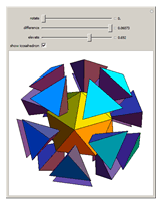The clear mirror pauses for us to catch our breath and absorb all these interrelated connections between the primal truths of the universe as it adds another twist literally. It shows us a golden tetrahedron based on Phi (φ). Then there are 20, one for each of the 20 cubes of the 210-cubit high Tower of Truth.

The 20 Golden Tetrahedra then gather around in a sphere and join to form a regular icosahedron, the 20-sided Platonic solid.

The twist comes in the form of a rotation, a necessary hyperspace rotation along the axis of the Tetrahedra and the icosahedron if the 20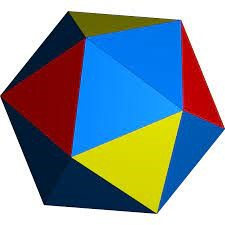component Tetrahedra are regular and must share faces and edges. That rotation is 22.2o computed as ArcCos (φ2/22), taking us back to (6000222) = 5778 and the 222.5o Phi (φ) angle. Once again, the Phi (φ) shift is a fundamental primal truth in the universe and Spherical Time. Thanks to the shift, around every vertex there are 20 regular 3-d Tetrahedra, 20 Triplets.

The clear mirror then advises us that the Inner Cosmos is pleased with our progress and that the Creator saw things as “good (טוב)” of numerical value 17, seven (7) times in Creation and that 17 is the frequency of 27.5/φ, and moreover that 17 = (27.5212/42).

Additionally, since the complete value of “good (טוב)” is twice 17 or 34, those 7 times that G-d found His Creation “good” have a complete value of (7 x 34) = 238, or 1/42 and (238 + 42) = 280, the value of the 5 final null judgements concealed within the 22 Names of Creation.

### It is Elementary

The clear silvery pool then throws us for a loop and shows us the Essential Cube of Creation with the central position 14 replaced by the 14th Element by mass, Silicon (Si), of atomic weight 28.086…., the element at the center of Man’s digital and clean energy technology, which by the way is not produced by melting sand as the Titans that control technology would have you believe. It is produced by mining quartz and fusing it with coal using enormous amounts of fossil fuel energy and with tons of CO2 as its waste byproduct.While certainly thought provoking with its connections to 28, 280, and 86 (Elohim), if we were not in the Inner Cosmos, we would dismiss this periodic table connection as mere coincidence. That is until the clear mirror shows us that the sum of the first 14 Elements is 215.330. The 330 can be reflective of the base measure (330 cu of 27.5”) of the encasing pyramid around the Tower of truth, but the clear mirror has other thoughts on the matter.  There are 33 letters in the 8 words of the 11 Triplets and the square root of the sum of the first 8 factorials (1! + 2!…8!) is 215.018, but more significantly the sum of the 6 Elemental Matrixes that form Zeir Anpin and create physicality (72, 27, 42, 48, 11 and 15) equal 215.

All well and good, but what of the rest of the 14 elements?

Hydrogen takes the #1 position with an atomic weight of 1.00784 as in value 784 or 282 that is baked into the Torah as the Inner Cosmos has painstakingly just shown us.

The second position, Helium (He) has an atomic weight of 4.00260, as in 22 or 202 and the YHVH (יהוה).

Then while the 7th element, Nitrogen (N) has an atomic weight of 14.00728, and is the 7th most abundant element in our galaxy, the sum of the 7 first elements (Hydrogen, Helium, Lithium (Li), Beryllium (Be), Boron (B), Carbon (C), and Nitrogen) have a cumulative total of 57.790, as in the year 5779 (2019 CE) as repeated 3 times in the first 1000 digits in Pi, or One (1) more than the event horizon and Spherical Time radius of 5778, also found there 3 times. As spooky as that it, we have to ask: If Man randomly named those 7 first elements, how come the first 4 initials (ההלב) equal 42, and the next 3 (בכנ) equal 72.

The Hebrew speakers among us realize that these are not the actual names of the elements in Hebrew, but they are reminded that these elements were not originally named by Israelis and not sourced Biblically either, or that their Symbols even in Hebrew go by the English letters. Meanwhile, the complete value for the 7 initials of the 7 elements is 165, or (6 x 27.5), the Primal Frequency and the 6-cubit width of the walls of the Holy Temple, the small gematria0 value of the 42-Letter Name.

The 6th element, Carbon (C), has an atomic weight of 12.00 as in the 12 Moons of the 6 ellipses of the Cosmic Wheel, while the average atomic weight for the first 27 elements, matching the 27 positions of the Essential Cube of Creation, is 29.5, as in the 29.5 days in the lunar month of the Cosmic Wheel. The average atomic weight for the first 6 elements is 7.29 or 93, as in the 729 value of the 6th level up (Gevurah) of the 42-Letter Name Matrix.

With the total atomic weight for the first 10 elements being 112.9… and their average being 11.29… we relate this to the 112 Triplets. We then relate the Triplets total gematria, (17,00035), to “good (טוב) or 17” times elef (אלף), 1000 or One, Binah, minus the complete value of “good (טוב). Another way we understand this now is that the 112 Triplets equal 1000 x (27.5212/42).

Not long ago the clear mirrored pool showed us a connection between the 1820 YHVH (יהוה) in the Torah and the 3 levels of the Blessing of One and in the 3 levels of the 42-Letter Name that equal 1820 to the Prime unicorn Number with 15,601 digits, as in the 1819th Prime Number. It showed us that 15601 is an emirp with 10651, the 1299th Prime Number and the 267th emirp. We connected it on our own to 1300 or 13 and the clear mirror added that 10651 is the 625th additive Prime Number because the digits in 625 sum to 13, the 6th Prime Number. This is always significant because 625 is the square root of the total words, letters, and verses in the Torah.

It now adds that 15601 is the 863rd emirp and that (863 + 267) = 1130, as in the sum of the first 10 physical elements, 113.

### 6 of 7

Beneath the swaying palms, our thoughts are interrupted, and we are shown that the way the Creator saw things as “good (טוב)” is presented 6 of the 7 times as “(כי־טוב)” like the 6 of 7 Days presented as (יוֹם) with the 7th (ביוֹם) having a sofit value of 618, representing 1/Phi (φ). It is also as in the 6 of the 7 days being accompanied by the phrase, “And there was evening and there was morning (וַיְהִי-עֶרֶב וַיְהִי-בֹקֶר)” whose gematria katan is 42, and whose numerical difference between evening and morning is 30, as in Ki (כי).

It is also like the 9 out of the 10 times that Vayomer Elohim (ויאמר־אלהים)” of numerical value 903, as in the 42nd Triangular Number, is presented without the additional Lahem (להם). And that the sum of the final letters in those 9Vayomer Elohim (ויאמר־אלהים) is 7200.

The single time Vayomer Elohim (ויאמר־אלהים)” is presented with the additional Lahem (להם) of numerical value 78 in the middle is on the 6th Day in the 6th paragraph in verse 1:28. It reminds us of the E8 Magic Star with the 78 dimensions in the center surrounded by 28 dimensions on each of the 6 vertices.

The clear mirror thought we would have noticed by now, especially as we count the Omer that many of the core Torah numbers (37, 73, 127, 137, 173, 27, 72) either include the number 7 or a multiple of it.

Day One is (1 x 7) = 7, like week 1 of the Omer. 2 is 14; 3 is 21; 4 is 28; 5 is 35, and 6 is 42. Altogether the first 6 days equal 147, the age of Jacob, who represents Zeir Anpin.

Everything has a purpose and a place.  The 7 can connote Malchut, but if you understand, it more often denotes Da’at (474), which permutes into Adat, assembly, as in the whole group.

While 6 x 7 = 42 and connotes the structure of the 42-Letter Name Matrix, when they are added they equal 13, One. While the Names of the letters Vav (ויו) and Zayin (זין) add up to (22 + 67) = 89, as in Chanukah that occurs 89 days after the Day of Creation, when they are multiplied, they equal 1474, or One and Da’at.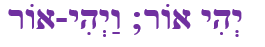Altogether the first 6 days equal 147, the age of Jacob, who represents Zeir Anpin, as in the 6 letters (יהי־אור) of “Let there be Light” on either side of the firmament (ו). When spelled-out the 6 letters (יהי־אור) have the numerical value of 702, Shabbat (שבת), the 7th day, that is found 147 times within words within the Torah.

### 4 Cornerstones

The clear mirrored pool then shows us the 9th row of the 72 Names whose sum is 702 and tells us that its first Triplet (דמב), the cornerstone of the 72 Name Matrix, is a permutation of the acronym for Moshiach Ben David (מבד). Moreover, the two cornerstones in this bottommost row in small gematria add up to (442 + 464) = 906, the sum of the bottommost row of the 42-Letter Name Matrix.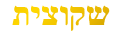In case we were wondering, the 4 cornerstones of the 72 Triplets summed together with the kolel equal (656 + 254 + 442 + 464 + 4) = 1820, as in the network of 1820 YHVH (יהוה) in the Torah and hyperspace. The top two cornerstones or bookends of the 9th Candle (first row) equal 910, which is half 1820 and twice 455 in the Path of One.

### 4 Aspects of the Name Ehyeh (אהיה)

The clear mirror further informs us that the sum of the two “Lights” from verses 3 and 14” is 848 or twice 424, Moshiach Ben David.  The Light is “Good” so 314 not only relates to Pi, Metatron, etc. but also to (3 + 14) = 17, tov, meaning “Good.” It is the value of the first cornerstone of the 72 Triplets (והו).

The clear mirror insists that from now on we cannot forget that 17, what the Creator saw, is 27.5/φ, and also that 17 = (27.5212/42), so the relationship to 314 makes sense in that 3142 is the value of the higher Name that incorporates both 21 and 42 and found at Exodus 3:14.

The still small voice interjects, “Everything is connected if you want to Understand; if you Understand, everything will be revealed.”

The letters are projections of their Names, and those 22 Names are projected from the hierarchy of the Names of the Creator.  The filled-in or spelled-out Names have more power, or you might say authority, in them as they are closer to the Source.

The 3 aspects of the Name Ehyeh (אהיה) add up to 455, but there are on occasion times when it is appropriate to use 4 aspects of the Name Ehyeh (אהיה), especially when aligned with the 4 aspects of the YHVH (יהוה).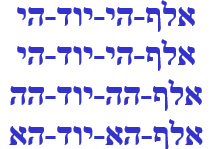When the 4 aspects of the Name Ehyeh (אהיה) are accessed their combined numerical value changes from 455 to (455 + 161) = 616, that of H’Torah, The Torah (The Laws). When the kolel (4) is added, they equal 620, as in Keter and the 620 letters of the 10 Commandments. The clear mirror adds that its counterspace is 380, as in Mizraim, Egypt.When the 4 aspects of the Name Ehyeh (אהיה) are aligned with the 4 aspects of the YHVH (יהוה), totaling 8 Names as in the 8 vertices of the E8 Lattice, their total is (616 + 232) = 848 or (2 x 424), Moshiach Ben David.

### The Secret of E8 and Abraham

The clear mirror adds that when the 8 vertices are added the average is (848 + 8)/8 = 107, as in the 107th Triangular Number, 5778, the Spherical Time radius. It further explains that the 248 dimensions found in the E8 Lattice are found in the Name Abraham (אברהם) whose standard numerical value is 248, and whose sofit value is 808, and whose full sofit value is 848.

The Name Abraham (אברהם) means Exalted Father. Abraham lived 175 years, and his Name (אברהם), established by G-d, is first utilized in the Torah in Genesis 17:5. This is the first of 175 times it appears in the 248 columns of the Torah.

Of those 175 times, 139 are without prefixes, as in the 5 Essential Sets of Triplets of Creation (14 + 72 + 27 + 11 + 15) = 139.

Of those 175 times, 27 have the prefix Lamed (ל) of numerical value 30, giving (לאברהם) the numerical value of 278, that of Ohr HaGanuz, the Light of Moshiach. Moreover, the complete value of the Lamed (ל) is 42, as in the 27 positions of the Essential Cube of Creation of 42 that align with the 27 times the prefix is used.

Of those 175 times, 8 have the prefix Vav (ו) as in the 8 vertices. Their total value is (8 x 6) = 48, as in the 6th Essential Element, the Matrix of 48 Letters of the 12 YHVH (יהוה).  These are also as in the 8 corners of the Essential Cube of Creation that add up to 112, aligned with the 112 Triplets of Creation.

Of those 175 times, One (1) time it has the prefix Mem (מ). The 3 prefixes (לומ) add up to 76, the ordinal value of Bereshit, the Torah’s first word, and the ordinal value of (לומ) is 31, giving them a complete value of (76 + 31) = 107.

### The 4 Spiritual Elements

The first 7 times the Utterances “Vayomer Elohim (ויאמר־אלהים) are presented in the Torah are matched with Light, Sky (Firmament), Water, Earth, Lights, Water, and Earth again, as in the 4 spiritual elements (Fire, Air, Water, and Earth), the 4 pressure mediations. The 6 dimensions of Zeir Anpin were established through Fire, Water and Earth, each twice, with the Firmament of Da’at, the 7th, over it. Those 7 elements of the 7 Utterances total (2010 + 7) = 2017, as in the square root (20.17424) of the Torah’s 6th word, of numerical value 407, and as in the atomic weight of the 10th physical element, Neon, 20.17.

The physical does not exist without the spiritual.

And just as those 9 Utterances “Vayomer Elohim (ויאמר־אלהים) equal 7200, the sum of the ordinal values of the 8 candles that equal 8000 within the 72 Names is 2017.

The clear mirrored pool then goes on to show us that the average of the two “Lights” with the two “Earths” is (848 + 592)/2 = 720, as in the 720 Lights in the 72 Names whose 72 square roots sum to 720.0, as in the 9Vayomer Elohim (ויאמר־אלהים)that equal 7200, which can be said to represent the 9 Candles of the 72 Names.

The silvery mercury in the still smooth pool sloshes around slowly in a soothing spiral until it is revealed that the gematria of the two “Lights (אור) and (מארת)” represent 42% of the 2010 total value of the 4 elements. The two “Earths (הארץ)” represent 29.5%, as in the 29.5-day lunar/Cosmic month, while the two “Waters (המים)” are equal to exactly half one “Air (רקיע)” making each Water ¼ of the Air (Firmament) that divides between the Waters above and below, as in the river of Gan Eden that is divided into 4 in verse 2:10. Together, the two “Waters” and the Air/Firmament equal 28.358% of the 2010 total gematria value.

According to the Zohar, there are 4 primordial elements connected to the 4 directions (N, E, S, W): Fire (אש), Air (רוח), Water (מים), and Earth (עפר). They total 955, and Man of numerical value 45 is introduced in verse 26 on the 6th Day, as the 8th Utterance. Man combines the 4 spiritual elements with the physical ones and when we add Man/Adam (אדם) to those elements the total is (955 + 45) = 1000. The ordinal value of those 4 elements is 145, like the 145 digits from the numerical string …1000… within Pi to the 1000th digit in Pi. Their total is 666, representing Zeir Anpin. Therefore, the complete value of the 11 letters in the 4 primordial elements is (955 + 145) = 1100.

Therefore, the average of those 4 elements is (1100/4) = 275, the Primal Frequency that runs through them. The clear mirror takes this opportunity to tell us that the word value 10 is found 11 times in the Torah for a total value of 110, merging the 10 and 11 dimensions of the Tree-of-Life and illustrating the first convergence point of the two primal frequencies (1.1 and 27.5 Hz).

This is the reason healing was reserved for the 5th Day, because the 4 spiritual elements had to be established first: Light, Sky (Firmament), Water, Earth.

The value of the first 7 Utterance elements, 2010, and the value of the 7 supporting words that come between “G-d Said,” and the element itself, such as “Let there be…” is 2005. When they are added with the 7 times the Utterance “Vayomer Elohim (ויאמר־אלהים)of numerical value 903, the 42nd Triangular Number, and with the 7 elements are revealed, the total for the 28 words of the first 7 Utterances is 10,336, as in the 336 letters in the 112 Triplets of Creation.

Moreover, the first 7 Utterance elements are found at verses 3, 6, 9, 11, 14, 10, and 24, which total 87, as in the value of “I am G-d (אני־יהוה),” while the number of letters in the 7 elements is 26, as in the YHVH (יהוה).

Since there are 27 letters in their 7 support words, the total for the 7 phrases of Creation (87 + 26 + 27) = 140. Nonetheless, when the values of those 14 elements and support words plus their verses and letters (140) are added together, (2010 + 2005 + 140), and when Man/Adam (אדם) is added from the 8th Utterance, just as the Inner Cosmos did with the 4 spiritual elements above, we get exactly 4200. Moreover, the same sum of the verses and letters for the first 8 Utterances is (30 + 113 + 30) = 173, the 42-Letter Name, and as we just learned 113 is the sum of the atomic weights for the first 10 physical elements that end with Neon, 20.179 or 20.18. No wonder it glows so bright.

### The Frequency of the 7 Days

The clear mirrored pool then explains that each Day is permeated with the Primal Frequency 27.5 Hz, with One cycle on Day 1, 2 cycles on Day 2, etc. The same holds true for every week of our lunar year within the Cosmic Wheel, especially the Cosmic Ring for the 7 Days.

The first Day or day is the purest single cycle of 27.5 Hz, and each subsequent day adds a cycle and a cumulative frequency, so the 2nd Day/day has a frequency of 55 Hz and a cumulative frequency of 82.5 Hz.

The 3rd Day/day has a frequency of 82.5 Hz and a cumulative frequency of 165 Hz.

The 4th Day/day has a frequency of 110 Hz and a cumulative frequency of 275 Hz, reflecting the first convergence point, and the full expression of the sefirot.

The 5th Day/day has a frequency of 137.5 Hz and a cumulative frequency of 412.5 Hz, reflecting the Phi (φ) angles at the center of Spherical Time and that day’s power to heal through harmonization.

The 6th Day/day has a frequency of 165 Hz and a cumulative frequency of 577.5 Hz, reflecting its relationship to the Tower of Truth of 8 base segments of 165 cubits and a height of 5775 inches, and of Zeir Anpin.

The 7th Day has a frequency of 192.5 Hz and a cumulative frequency of 770 Hz, meaning the cumulative Light on the 7th Day is equal to 7 times 110, the convergence point, and the full expression of the sefirot.

### A Special Blessing by the Creator

The air pressure suddenly feels different. It is that uneasy feeling before a storm, yet there appear to be no clouds on the horizon, or anywhere for that matter, just a sense of pressure unfolding, as if maybe time was running out. The clear mirror has a hurried feel in the way it is displaying the insights to us.

We have most recently learned about the connection of the Divine summation path (11391455 1820) sourced from One (1) to the Blessing of the Cohanim and the connection of (1 + 13 + 91 + 455 + 1820) = 2380 and that (2380 + 1820) = 4200.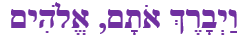Now, the clear mirror takes us one step deeper and explains that not only is 1/2380 = 42, but in the first 3 words of Genesis 1:28 the word for “and Blessed (ויברך),” as in “G-d blessed them,” referring to the men and women G-d created when He created Adam, has the numerical value 238. The word used for them, otam (אתם) is a permutation of emet (Truth) of numerical value 441. It also wants us to know how deep this truth goes, by explaining that the sofit value of this 3 word Blessing of the Creator with the kolel of 3 is 2368, the year Moses was born, the 26th in the lineage of Adam, and as we saw earlier, Moses (משה) of numerical value of 345, is the numerical value of the sum of the Triangular Numbers associated with the Hebrew letters applied to Emet (אמת) and thus (אתם), or (1 + 91 + 253) = 345. G-d blessed them with Moses, blessed us with Moses, and blessed us all with the Name of Healing (מהש).

As a bonus, it is explained to us that they were created in perfection, and in the state of Binah. In Genesis 1:27, just before the aforementioned Blessing, we learn that G-d Created him, male and female (ברא־אתו־זכר־ונקבה). These 14 letters begin with the first Triplet (ברא) in the Torah and contains the permuted 6th word of the same verse (אתו) and also contains male and female (זכר־ונקבה) of joint numerical value 390, as in the 5th word of the Torah, “Heaven,” making the full value of the phrase exactly 1000, and thus created in the image of Binah, completion.

Before continuing the clear mirror further explains that the Torah’s 5th word (השמים) that not only includes the Name of Healing (מהש), the 5th of the 72 Names and 45th overall Triplet, but as written is (5,390), as in the sum of the cumulative frequencies of the 7 days, 5390 Hz.

### The City and a Tower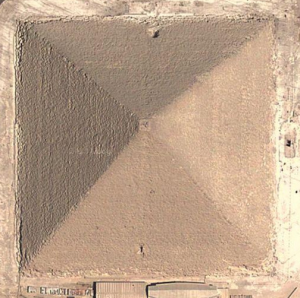It is also telling us to put in the back of our heads that 5390110 (the convergence point) = 5280, one mile, and that 110/5390 = .020408 is not a coincidence.

We accept that and figure the Inner Cosmos has something special in mind for us, especially in respect to the promise made by the still small voice at the beginning of this day, but what the clear mirror shows us next is a little weird, and being on a journey through the Inner Cosmos that is saying something.

Those cumulative frequencies of the 7 days, 5390 Hz are equivalent to 5390 inches which equal 449.1667 feet, which are 32.0833 feet shy of the 481.25-foot height of the Tower of Truth and its encasing pyramid. What is weird is that 32.0833’ is equivalent to the encasing pyramid’s capstone base, whose height is 20.42 feet.

We are then reminded that the capstone’s base 32.0833/756.25 feet is 4.24242% of the encasing pyramid’s actual base, and that 20.416625/481.25 = 4.24242% of the encasing pyramid’s and Tower’s actual height.

And if that were not strange enough, the encasing pyramid’s capstone base measure of 32.0833’ is exactly 1/10th the 320.833’ from the center of the Holy of Holies to the center of the Central Altar of the Future Holy Temple,

The clear mirror further explains that the center of the Holy of Holies to the Eastern edge of the Outer Courtyard is 296 cubits, analogous to the numerical value of the Torah’s 7th word, Earth (הארץ), and that the ratio between the word Tower (וּמִגְדָּל) City (עִיר) from Genesis 11:4 is 83/280 = .29642.

Could the City and a Tower (עִיר, וּמִגְדָּל) be the Tower of Truth and the encasing pyramid? The gematria of City and a Tower (עִיר, וּמִגְדָּל) is (280 + 83) = 363, H’Moshiach, and the ratio of the height/base of the encasing pyramid, the Tower to the City is .6363.

Returning to the special blessing, it is explained that the 4 final letters in “G-d Created him, male and female (ברא־אתו־זכר־ונקבה)” spell out the “The Light (האור), which equals 212. The initial values of male and female (זכר־ונקבה) equals 13, echad, One (1) and match the (2 + 6 + 5) of the 2nd, 6th and 5th words of the Torah’s first verse embedded in the full phrase.

They form the 3rd triplet in Pi (3.14159265358…), just before 358, Moshiach, the 4th triplet. Meanwhile, the 4 final letters in (ברא־אתו־זכר־ונקבה) that equal 212 represent half of the two middle triplets (159 + 265) in Pi (π) that equal 424, Moshiach Ben David.  It takes two (2).

While it is at it, the clear mirror adds that the two words “He Created them (ברא־אתם)” also in Genesis 1:27 between the phrase above and the referenced Blessing on “them,” have a complete numerical value of 703 as in the 6th and 7th words (ואת־הארץ) in the Torah’s first verse, which is analogous to the 6th and 7th letters (ו־ז) in “G-d Created him, male and female (ברא־אתו־זכר־ונקבה)” being of value 6 and 7. Naturally, we note that (6 x 7) = 42 and that (6 + 7) = 13, the One Source, 42.

It does not take a genius, just Understanding.

The clear mirror adds that verse 42:13 contains “The One (והאחד)” referring to Joseph, as does verse 42:32. Joseph is the One person named twice albeit clandestinely (האחד־והאחד), as in “His Name is One” echad ushmo echad (אחד־ושמו־אחד)” that contains the central word Ushmo of numerical value (2 x 173), the small gematria of the 42-Letter Name.

### It is Always and has Always Been About the One

The clear still pool reflects the first 13 physical elements and their 13 atomic weights, and it multiplies them together into a rather long number: 42442387397160.4090….

The product of the first 13 atomic weights equals 424, 424, or Moshiach Ben David twice, or alternatively 424, 42 and 1/42!?!? And their sum is 187.2446, as in the 187 chapters in the Torah, and as in the 17350-digit palindromic Prime that peaks at 2446.Speaking of palindromes, which along with Triangular Numbers are a favorite of hyperspace mathematics because of their symmetry, the sparkling sliver pool advises us that there are only 28 palindromic Triangular Numbers less than 1010. They include, 55, 171, 3003, 15051 and also 6, 66, 666, and 66066, the 363rd Triangular Number, and the 13th palindromic Triangular Number.  It is always about One, and 66066 is the sum of the 363 Days in the Cosmic Wheel that control the 6 dimensions of Zeir Anpin.

### There was Morning and There was Night

We are still trying to comprehend a population of male and female that the Creator blessed to be fruitful and multiple before He even Created Eve from Adam, as the clear mirrored pool shifts back to the 7 Days plus the 6 times the phrase Let there be Light (וַיְהִי-עֶרֶב וַיְהִי-בֹקֶר) was used to describe them. It has a total gematria katan of 425, or 424 + One (1) and a complete gematria of 1424 or One (1) plus 424, Moshiach Ben David, which is why the 7 Dimensions intwined in the Essential Cube of Creation is known as the Bird’s Nest, the concealed vessel of Moshiach Ben David. As we know, the value 1424 is also Kadosh Kadoshim, the 203 Holy of Holies.

“There was morning (וַיְהִי-בֹקֶר)” has the numerical value, 333, the same as the darkness (הַחֹשֶׁךְ) that the Creator divided from the Light in verse 4 of the Torah. In the 5th and very next verse we have, “And God called the light Day, and the darkness He called Night. And there was evening and there was morning, one day. (וַיִּקְרָא אֱלֹהִים לָאוֹר יוֹם, וְלַחֹשֶׁךְ קָרָא לָיְלָה; וַיְהִי-עֶרֶב וַיְהִי-בֹקֶר, יוֹם אֶחָד).

“And there was evening and there was morning (וַיְהִי-עֶרֶב וַיְהִי-בֹקֶר)” literally describes the passage of night, from sunset to dawn, not a nighttime and a daytime. There was evening, darkness. The light was called good, not the morning.

### The Secret of the 2 Paths

G-d Created him, male and female (ברא־אתו־זכר־ונקבה). Is this the secret to the Two (2) Paths to One?

The initials and final letters of the 2 words male and female (זכר־ונקבה) spell out “raz (רז), secret” of (וה), representing Zeir Anpin and Malchut of the YHVH (יהוה). Their value of 390 alludes to Heaven (שמים) within the Torah’s 5th word (השמים) and to the 390,625 or 58 words, letters, and verses in the Torah. Where there is space there is counterspace. It is not positive and negative; it is hyperspatial physics. The two Paths are not separate but must work in concert as One. They were created as One and must return unified. Counterspace and space do not fight one another; they coexist and balance one another, nor do they covet one another.  This is what the 10 Commandments are about.

# 8 Mysteries Revealed

Through the palms we see a ring of fire surrounding the oasis, a dancing bright intense blue veil, white hot in spots and in waves. No one gets in or out. None of us moves. Our focus intensifies on the clear mirrored pool reflecting the undulating flames in a ring around its outer edge.

### The Day of Creation

The still small voice explains that 625 is not only the square root of the 58 words, letters, and verses in the Torah; the 25th of Elul is the 25th Day of the 6th month or 625. It is the Day of Creation, the 258th Day of the Cosmic Wheel.

When the solar calendar of 365 days is imposed on the lunar/spiritual Cosmic Wheel, the Day of Creation, the 258th Day becomes 107 days from the end (365258) and all becomes tied to the event horizon in 5778.

That the Creator of the Torah and of the Cosmos also Created the elements to populate Zeir Anpin and that He would make them all coordinate and integrate is no surprise; in fact, it is only logical. But what about these harmonics of the frequencies?

The Creator did not embed these harmonics into Phi (φ) and into the Primal Frequency (27.5 Hz) to impress us. It was specifically embedded in the 7 Days of Creation for us to get closer and more harmonious with the Source.

### The Frequency of Creation

The clear silver pool shows us Noach’s ark, and reminds us that the animals, birds etc., did not just enter the ark 2 by 2. They were specifically grouped sometimes in pairs, sometimes in 7’s.  The exact configuration gives us a digital string of 22727.  It then reminds us that 22727 is the difference (424,000401,273) between full Moshiach Consciousness and the boundaries of the Torah, the 5 Core quantitative Elements (words, letters, verses, rows, columns) of the Torah Matrix.

From there we are reminded of the self-referencing equation between the 22 and 27 letters of the Alef-bet, 27/22 = 1.22727. This reflects the exact initial sequence in the 22 verses of Chapter 6 of Bereshit and the first verses of Chapter 7 in parsha Noach: 300 – 50 – 30 – 1 – 2 – 2 – 7 – 2 – 7. After giving us the dimensions of the Ark, we are given the secret sequence.

The clear mirror explains that there are 22 letters and 7 of them represent the 7 Planets. There are 304805 letters in total in the Torah. Of them, 4805 are the letter Pe (פ). It is one of the 7. That leaves exactly 300,000 letters, analogous to the 21st letter, Shin (ש) of numerical value 300. There are 15,595 letters Shin (ש) in the Torah. When the 15,595 Shin (ש) are added to the 4805 Pe (פ), there are 20400 letters in total, representing the letter Caf (כ) and Tav (ת), or the Sun and the Moon respectively, also part of the 7 planets.

The 20400 naturally reminds us of the ratio 110/5390 Hz = .020408, the convergence point divided by the cumulative frequency of the 7 Days, both connected with the 5280’ mile. Also fresh in our heads is that 5390 inches is 32.0833 feet shy of the 481.25-foot height of the Tower of Truth and its encasing pyramid, the city, matching its capstone base, whose height is 20.42 feet.

The clear mirror adds a new revelation that those 32.0833 feet are equivalent to exactly 14 cubits of 27.5” matching and aligning the capstone with the central position in the Essential Cube of Creation and Spherical Time. While the height of the capstone is 8.90 cubits as in the 89 days from the Day of Creation to Chanukah, the two measures together, base and height, equal 22.9, as in the Frequency/Time ratio 27.5/12 = 2.29.

It then shows us the Spherical Time sphere with King David (14)’s midpoint in his life as an inner sphere with radius 2889 years in the center of the 5778-year outer one. While that year 2889 HC should have nothing to do with the year 2018 in either the Hebrew or Gregorian Calendar or the year 3760 (0CE) in the Divine Calendar, the image clearly shows us that because of Spherical Time it is equidistance between them both. There are 871 years between 2018 and 2889 and 871 years between 2889 and 3760, which is why in hyperspace the 2018 physical and the 3760 spiritual years are equal, and as we learned equated by the speed-of-light.

As the sphere glows brighter and brighter we are shown the volume of the 2889-yr core is 1.01002 x 1011 and that the volume of the full 5778-year sphere is exactly 8 times that, 8.08018 x 1011.

The clear mirror leaves no doubt that the connection to 11, the 11 sefirot and Da’at is purposeful and more than intentional. Then…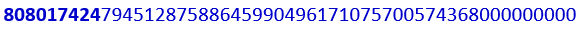It is the 80801742479451287588645990496171075700574368000000000 different symmetries in the massive Monster Group that is so baffling.  We covered a lot about it already, even its connection to Abraham whose sofit gematria is 808. But now we understand that its existence is tied to the volume of the 5778-year radius Spherical Time sphere, 8.08018 x 1011.  Of this there can be no doubt, as the two time frames, physical and spiritual, are separated by 871 and 871 = 1742 years, or (37602018) which end in 424, as in the Spherical Time circumference, 36304.24470

We then see…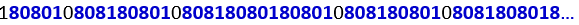The two strings 80801 and 808018 are repeated over and over again within the

palindromic prime of 1560 repetitions and 15601 digits.

It feels like the Inner Cosmos has been dropping breadcrumbs for us all along.

After its Spherical Time interlude that clear mirrored pool returns to the 22727-sequence in the Torah, where we see the dimensions of the Ark, 300 – 50 – 301 – 2 – 2 – 7 – 2 – 7. These total (300 + 50 + 30) = 380, as in ShinPe (שפ), the complement to 620.

Whether we utilize the 27 letter values or the 22, we get the same fractal results. The clear mirror shows us that when we divide the total sofit gematria of those 304805 letters by 7, we get 33640626/7 = 4805804.  This is the same 33640626 value whose square root is 5800.054.

### The Planck Diameter

The clear mirror once again takes us through the exact number of verses, words, and letters in the 5 Books of Moses being precisely 58, with the total gematria sofit—using the 5 final letters being (5800.054)2 which is also (5800.02 + 625), and that .625 = 5/8.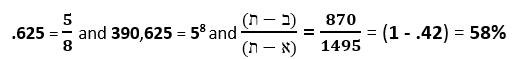As the sky above us brightens, as if the Sun were dawning in all directions at once, we are reminded that while the final letters of “Let there be light. And there was light. (יְהִי אוֹר; וַיְהִי-אוֹר)” has a numerical value 420, the full phrase has a complete gematria of 580

Speaking of final letters, we are then shown that this 5800-square root value for the entire Torah is equal to the difference between the sofit gematria values and the standard ones of the 22 Names, (10048 – 4248) = 5800, and that (580022) = 5778.

Moreover, that same total sofit gematria of the Torah whose square root is precisely 5800.054 works out to (112 x 300363),as in the 112 Triplets that occupy 336 days in the 363 Days of the Cosmic Wheel.  The average word value of those 304805 sofit letters with the kolel or (33640626 + 304805)/79975 = 424.450.

The clear mirror now shows us the Planck diameter h/π or 21.0914362859 x 1035 and reminds us that the encasing pyramid of the 210-cubit Tower of Truth measures precisely 580.0 feet of vertical height from the base of the Subterranean Chamber to the tip of the Pyramid, and that the Primal Frequency of the Planck’s Diameter is (27.5 Hz x 21.09143628 x 10-35) = 580.0145… It is not only connecting the height measures of the encasing pyramid/Tower of Truth with the Planck Constant and Diameter, but it is connecting them to the 22 letters of the Alef-bet through the values 5800 and 5800.0542 What is more is that 580.0 feet is 253.0 cubits of 27.5”, and 253 is the 22nd Triangular Number, the sum of the 22 ordinal values of the Alef-bet. While the Tower of Truth is 20 levels high, 210 cubits, its full height with the 2 underground foundation levels down to the Subterranean Chamber make its total height 253 cubits, 22 building blocks, 22 levels, and 22 stacked cubes.

As Abraham wrote in the Sefer Yetzirah 2:2,
Twenty-Two Foundation Letters:
He engraved them, He carved them,
He permuted them He weighed them,
He transformed them.
And with them, He depicted all that was formed
And all that would be formed.

While the 20 revealed levels in the Tower of Truth equal 44,100 cubits3 or 44,100 blocks in total, the 22 total levels occupy 64,009 blocks or 64,009 cubits3, (20,0009) more. The clear mirror points out that the 43 courses that are below ground equal 17.00% of 253 total levels/cubits, and 20.48% of the visible 210 courses, as in (2 x 1024) or 211.

When divided by the 210-word values in the Torah, we get (64009/1024) = 62.50 blocks for each value of the 1024-word values, as in the Day of Creation (625), H’Keter, and the square root of the 3 main Torah elements.

The clear mirror tells us that while 390,625 words, verses, and letters is 5 to the 8th power, and 1024-word values is 2 to the 10th power their quotient is 58/210 = 381.47, and right before the 22727-sequence in the Torah, there are the dimensions of the Ark, 300 – 50 – 301 – 2 – 2 – 7 – 2 – 7. These total (300 + 50 + 30 + 1) = 381.

### The Fine Structure Constant

The clear mirror reviews what we were previously taught about the Fine-Structure Constant α of of dimension 1, meaning it is simply a number and not dependent on other factors, and that it is approximately 1/137.035999… It is the “coupling constant” or measure of the strength of the electromagnetic force that governs how we perceive the interaction between electrically charged elementary particles (e.g., electron, muon) and light (photons).

A more complicated way to see it is as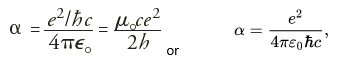where e is the charge of an electron, = h/ where h is Planck’s constant, where ε0 = 1/µ0c2 is the electric constant (permittivity of vacuum, also known as free space) and µ0 is the magnetic constant (permeability of vacuum), and where is the reduced Planck constant and c is the speed of light. This constant is proportional to the square of the coupling strength of the charge of an electron to the electromagnetic field.

Since the fine Structure constant =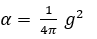The Gauge coupling parameter (g) is: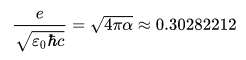It has been well established that the Torah’s first verse of numerical value 2701 and the 42-Letter Name of numerical value 3701 are integrated in numerous ways, but their first relationship to the Fine Structure Constant (1/137.03) is through the square root of 1370 = 37.01… and through the square root of 730 = 27.01…. While (1370 + 730) = 2100 and the total gematria of the Torah is 21009826, and while (1370 730) = 640, it is the ties of 730 to the higher dimensional math sum of the integers from 1 – 73 that equal 2701 and the ties of the 1370 to the Fine Structure constant’s 1/137.0… that are more significant.

The Fine Structure constant, 1/137.035999 is equal to .007297353… and while the entire 2nd line (קרעשטן) of the 42-Letter Name Matrix has a numerical value of 729 or (9 x 9 x 9), the 2nd Triplet (שטן) of that same the 2nd line has a value of 359 as in 1/137.035999.

Moreover, the small gematria of the 42-Letter Name is 173 and 1/42 x 1/137 = .000173… Yet perhaps most significant of all is that 42 times the Fine Structure Constant (1/137.0…) minus the coupling constant) or (42/αg) = (42/137.03599.302822131) = 11/3000 = 272.72727, as in the 27 Letters and positions of the Essential Cube of Creation.

Through the simple division of the Fine Structure Constant by the Primal Frequency (27.5 Hz) we get (1/137.03599)/27.5 = .00265358, as in the 3rd and 4th triplets in Pi (3.14159265358…).

We can align the digits simply by dividing the Fine Structure Constant by (27.5 x 102), which is the 100-cubit height of the Future Holy Temple. This allows for the equation (π – (1/137.03599)/2750 = 3.14195000000…., an exact 6-digit blank space or hole in Pi(π).

A slight modification in the calculation of the Fine Structure Constant as 1/137.035671756… would work out to exactly Pi. In other words, the difference between them would be exactly 3.14195. Do we have the right number for the Fine Structure Constant? Before it was changed to an uncertain 1/137.03599, the Fine Structure Constant had an established value of 1/137.0356+ just 2 years ago.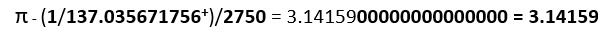In other words: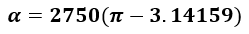There are several different ways to calculate the Fine Structure Constant, most of which includes calculating Pi(π), Planck’s constant, the speed of light, and the elementary charge. Some also include calculating the magnetic constant, electric constant, and the coulomb constant. There are still other complicated ways: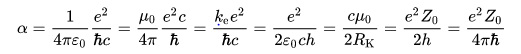Yet, all that is needed is a knowledge of Pi (π) and the Primal Frequency:The clear mirror adds that when we divide both Pi(π) and Phi(φ) by the Primal Frequency (27.5 Hz) we get 0.114276096 and 0.0588376 respectively. Then when we add them and take their inverse the result is (1/0.173113696) = 5.77655, as in 5777 HC.

The clear mirror adds that 1/.00265358…. = 376.847997588 or 376.848 with 376 being the value for Shalom (שלום), peace, and associated with the 3760 years of Spiritual Time, and 848 being (2 x 424, Moshiach Ben David) and the sum of the two fully expressed Names:And with the 2 Triplets 265 and 358 being the 3rd and 4th Triplets in Pi, we are told that with the kolel they equal (265 + 358 + 2) = 625, as in the Day of Creation again, and moreover that the 4 Triplets (314 + 159 + 265 + 358 + 4)/4 average 275, the Primal Frequency.

The clear mirror adds that the first 2 Triplets (314 + 159 + 1) with the kolel (1) equal 474, Da’at, and the middle two (159 + 265) equal 424, Moshiach Ben David, with the middle digits in all 4 triplets (314 , 159 , 265 , 358) spelling out 1565, the YHVH (יהוה).

The 8 digits in the first and third digits (3123 + 4958) = 8081 as in the 1560 times it is repeated in the 15601– digit Prime Number unicorn, and the clear mirror informs us that altogether, the three 4-digit strings equal (10,000354), as in 105 less the 12 lunar Months (29.5 days) in the Cosmic Wheel.

With so much to absorb, the clear mirror pool adds that in case it was not clear, the equation, 1/.00265358…. = 376.847997588 is derived from (137.0356 x 27.5) = 3768.47997588 with 79975 being the exact number of words in the Torah.

With the connection to the 72 Triplets in the Planck Diameter (21.09143628 x 10-35) through its value of 9143, we wonder whether the 3599 in the Fine Structure Constant (1/137.03599) reflects the 9359 value of the 72 Triplets with its kolel for the 216 letters.

The clear mirror reminds us that the Fine Structure Constant is based on Planck’s constant and simultaneously on Pi (π) and the Primal Frequency (27.5 Hz), and that Planck’s constant was designed to interact with the speed of light. These are all just the results of the interactions of the primordial hyperspatial fields.

### The Speed of Light

The 5778-year radius of Spherical Time is comprised of the two simultaneous time frames, Spiritual and Physical, of 3760 and 2018 years respectively. As we know the relationship between these two is the proportion of the speed of light, 3760/2018 = 1.86282, as in 186,282 miles per second.

Referring to the speed of light, the clear silver pool shows us that (200,000186,282 mps) = 13718. Then with shifting decimal places, we are shown that 1/729 = .001371742, and that 1/137 = 729927 and that 1/.001371742 = 729.

We are being shown that the Fine-Structure Constant (1/137.0) is connected to the number 2 or 20 and to Spherical Time though the speed of light (186,282 mps).

It is then revealed that 1742 in .001371742 is the gap in Spherical Time between 3760 and 2018 years bridged by the speed-of-light ratio, and we know that 137 means “parallel.” It is a reminder that the Light is Good (17) and that 42 is the Source.

And yes, we have just seen 1742 appear in the Monster Symmetry Group 80801742…, which prompts the clear mirror to tell us that Noach, the 10th generation was the 601 years old at the time of the Flood.

It seems as if it must have skipped something, but it waited until one of us figured it out. The 196,883 dimensions of the 54-digit Monster Symmetry Group less the speed-of-light 186,282 mps equals 10,601.

Free to move on, it further reminds us that the value 2 is an integral part of the Spherical Time equation.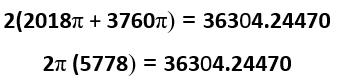Before moving on with its explanation about the speed of light, the clear mirror explains instead about the difference between 363, the value of H’Moshiach and 424, Moshiach Ben David, the key values in the Spherical Time circumference. Earlier, the Inner Cosmos explained to us the significance of Abraham, the exalted father, to the Torah, the Cosmic Wheel, and the 248 dimensions in the E8 Lattice. Now it is explaining that before the 175 times Abraham (אברהם) was mentioned in the Torah, before G-d gave him his Names, he was called Abram (אברם) and he was mentioned 50 times as is, and 61 times in total with the prefixes.

Those 50 times plus the 139 times that Abraham (אברהם) does not have a prefix equals 378/2, as in the middle of the 27 Positions/ordinal values of the Essential Cube of Creation where the letter Nun (נ) of value 50 is found, and as in “His Name is One” echad ushmo echad (אחד־ושמו־אחד).”

Nevertheless, 61 is the difference between 363, the value of H’Moshiach and 424, Moshiach Ben David in that (424363) = 61, as in Ani (אני), I am, the 37th Triplet at the center of the 72 Triplets. It then translates this hyperspace relationship into “(אני־המשיח־משיח־בן־דוד) I am H’Moshiach, Moshiach Ben David.”

From the Light of Moshiach, the clear mirror returns to the speed of light and repeats a complicated explanation it once gave us on this journey so deep into the Inner Cosmos.

In our physics, a frequency is the distance between two wave peaks and the higher the frequency the closer together those peaks and thus the shorter the distance. That pretty much defines the entire electromagnet scale as we understand it. At 27.5 Hz the corresponding wavelength in space, meaning one solely contingent on the speed of light, is 6774 miles. A single wavelength at 27.5 Hz is 67.20% of the earth and moon diameters together.

This means that the speed of light/1 cubit, or 1 cycle is 186,282 mps/27.5 = 6774 miles.

Measuring things by the number of cycles of a specific frequency is akin to applying modular mathematics to determine how many full cycles and their remainder or partial cycle. When Mod (27.5) is applied to the Fibonacci numbers, it has a repeat cycle of 20, as in the 20 levels of the Tower of Truth and they total 165, as in the midpoint, 165 cubits, scored into each of the 4 bases of the encasing pyramid.

That is certainly interesting, but the clear mirrored pool wants to remind us of what happens when we take Mod (27.5) of the set of all integers.

As we have previously explained, the numerical values of the 27 letters of the Alef-bet are based on a simple exponential equation and a sine wave that rides astride it, yet they are also 3 parallel groups of 9: 1 – 9, 10 – 90 and 100 – 900. It is because of that structure that the 27 letters sum to 4995. There is another way to get to 4995 though, and it involves the uniqueness of the Primal Frequency (27.5 Hz).

Decimal places within numbers are just multiples or divisions of 10x so we can basically ignore them.  So, considering the set of all whole numbers individually as our numerator, we can divide any of them by another whole number (the denominator) and the results can either be another whole number, an irrational number, or a repetitive pattern. Only a tiny set of them will result in 2-digit repeats that sum to 9, as in .090909…, .181818…, .272727…, .363636…, .454545…, .545454…, .636363…, .727272…, .818181… and .909090….

The sum of those resultant remainders (.090, .181, .272, .363, .454, .545, .636, .727, .818, and .909) is 4.995, as in the sum of the 27 letters of the Alef-bet.  If the decimal place is extended indefinitely as happens naturally, the result is 4.9999… = 5, the number at the core of the Torah (58) and of Phi(φ), which is based on the square root of 5.

There are only a few such numbers that can be used as denominators, the active part or frequency of the equation, to generate such results. They are 1.1, 2.2, 5.5. 11, 22, 27.5 and any multiple of 27.5 in the order of F(x) = 27.5(n + 2). At first glance that gives us exactly 8 frequencies through 110 and of them the number 22 stands out as reflective of the 22 Hebrew letters and the simple equation (27.55.5) = 22.  Yet on further reflection, we realize that F(x) = 27.5(n + 2) yields 55, 110, 137.5, 220, 275…, meaning that 1.1, 2.2, 5.5. 11, 22 are just derivative of 55, 110, and 220. Therefore, it is only by dividing a number by the unique frequency 27.5 Hz or certain of its even number derivatives that we can generate strings of numbers with repeating sets of 9’s.

Not every number that we divide by 27.5 will yield these special results based on 9.  The exception are the numbers that are derivate of 27.5 such as 1.1, 2.2, 5.5. 11, 22, 27.5, and any multiple of them, which will naturally yield whole or terminal numbers.

As it turns out, each of these special derivative numbers are exactly 11 units apart. And every grouping of the 10 results between them ends in a complete set of repeating .363636…, .727272…, .090909…, …,.454545…, .818181…, .181818…, .545454…, .909090…, .272727…,  .636363… in that exact order, forever.  The clear mirror shows us the Tree-of-Life of the 11 sefirot including Da’at.

For what it is worth these are self-organized into 5 concentric pairs of 99: (.36 + .63), (.72 + .27), (.09 + .90), and in the center (.81 + .18), like the 99 we were shown in the Magen David of 72 in the center of the 42-Letter Name Matrix.

The hidden pattern in these 10 endlessly repeating numbers that are responding to the frequency 27.5 is: (+36, -63, +36, +36, -63, +36, +36, -63, +36, +36) or simply (+36, +36, -63).

The results are the same whether we use the square roots of 36 or .36, so for simplicity, let us use 36, 72, 90, 45, 81, 18, 54, 90, 27 and 63.  The results in order: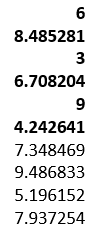And the sum of the results of the 10 square roots of those 10 resultant remainders order of 9, as in .090909…, .181818…,.272727…….909090 is 6.774…, matching the value for the wavelength of 27.5 Hz against the speed of light, 6774 miles.

The length of the wave created by the frequency 27.5 Hz is thus the sum of the square roots of its 10 digital residues. There should be no logical correlation in the physical realm between them unless those 10 residues relate to the speed of light and that the distance of 5280 feet/mile were baked in, which makes no scientific sense, unless the universe was intentionally designed that way, a harmonic balancing point between Light and light, the bond that ties all physics, mathematics, and metaphysics together.

So the speed of light, known as c in scientific terminology, and measured at about 186,282 miles per second is can be said to be based on the simple measure of the Primal Frequency, 27.5 Hz, and the simple numbers 110.

There is almost no aspect of our modern physics that is not dependent on the constant (c) for the speed of light. And yet, the universe was created so that it was based not on propagating waves or imaginary particles but on a single simple number, 27.5 and the simplest of base 10 mathematics, what we have previously studied as the 10 fields from 1 to 10.

The 186,282 miles per second is obviously dependent on the measure of the mile as 5280 feet as well, and just as 186,282 mps/27.5 = (6774 miles x 5280 feet), 5280/27.5 = 192, the number of hours in the crown jewel of the Cosmic Wheel, the 8 Days of Chanukah connected to Binah consciousness.

The 9th candle lit 8 times also equates to (8 x 24) Triplets = 192 Triplets and 192 hours of Binah consciousness. Moreover, those 192 hours of the 9th candle are not only equal in duration to the 8 candles but are equal in ethereal value to the full 72 Triplets, as 8 x 1143 = 9144 = 9143.

The clear mirror reminds us that within the 64 triplets or codons in our DNA, each base unit or nucleotide will appear 48 times and since there are 4 of them, (48 x 4) = 192 times that these 4 nucleotides are repeated in our 64 DNA codons (triplets). Since the 4 Hebrew letters (אכגת) assigned to the 4 nucleotides add up to 424, Moshiach Ben David they are aligned with the Spherical Time circumference (36304.24470) and the cube root (3.6342412) of 48.

When we add 424 to the 192 times that these 4 nucleotides are repeated in our 64 DNA codons (triplets) it equals (424 + 192) = 616, the value of “H’Torah, The Torah.”

The Primal Frequency (27.5 Hz) permeates the Light, the Torah, the Cosmic Wheel, and our DNA.  While a 3-d cube has 26 collective vertices, edges, and faces, connecting our existence to the boundaries of Zeir Anpin and the YHVH (יהוה); and the 4-d Tesseract hypercube has 72 collective vertices, edges, and faces; connecting the 72 Triplets to the first step toward freedom; the 5-dimensional Penteract hypercube has a collective 192 vertices, edges, and faces; and the 6-d hypercube has 192 edges and 64 vertices, likes our DNA.

As we just saw, the speed of light, 186,282 mps (miles per second) ties the sacred cubit/Primal Frequency (27.5 Hz) to the essence of the number 9, including the 9 Candles, throughout as delineated by the 10 sefirot (dimensions). This is the same sacred cubit that must be used in the building of the Future Holy Temple. It is the same concept of frequency at the core of Spherical Time.

Then in relation to Pi(π), the clear mirror reminds us that 9 divided by the small gematria of the 42-Letter Name is 9/.123049… = 73.141593… defining 70 and Pi(π) to an astonishing 7 degrees of accuracy. We have seen that a hundred times, but what was never revealed to us before is that the 2304 embedded in the 42-Letter Name’s first line/level is (5280*12)/27.5 = 2304, the exact number of cubits (27.5”) in the mile.

The Staircase of the Generations briefly appears before us as a mirage in the desert sands.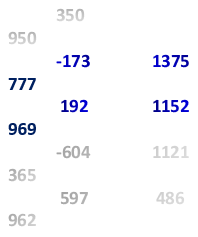The generations between Methuselah and Lemekh, the 8th and 9th generations are highlighted. We see that the Inner Cosmos are pointing out the 192-year differential and associating it with 5280/27.5 = 192, which is when we realize that the sum of the absolute differentials through those 192 years is 1152 and that 1152 is 2304/2 or half a mile in cubits (27.5”). And since the gist of the discussion is the speed-of-light the clear mirrors adds that the speed of light in astronomical units is 173 AU/day, as in the small gematria of the 42-Letter Name, and the 173 Keys to Heaven.

The Phi (φ) angle 137.5o is also illuminated, and then we notice that the absolute total of the 192 and 173 differentials equal 365, as in 365 solar days and Enoch’s 365 years before he was taken by G-d. The mirage evaporates.

We never thought to look there, as we did not think to look deeper beyond the Name (אלף־הא־יוד־הא) into the 143 associated with the 9 Candles and especially the 9th Candle of the 72 Names through its sum 9143, its first row, 1143, and its column average 1143, and the Triplet average 143 of the 9th Candle. We never thought there was a connection to the 1430648,809.35 cubits per part of a minute.

We also never realized the difference between H’Moshiach (363) and the complete value of Moshiach Ben David (506) as expressed in Da’at, the 11th sefira is (506363) = 143. Nor did we realize that like the sum of the Names of the 5 Books that equals 2480, the sum of the first word in each of the 5 Books of the Torah is 1430, whose square roots total 72.32721. And whose cube roots total 28.543…., which together total 100.870, reminiscent of the square roots of the 72 Triplets and of the 9 rows of Triplets that equaled 720.0 and 280.0 for a total of 1000.054.

The clear mirror points to 28.543… and points out that 2854 HC was the year King David was born, and that 32, 27, 72, 21 and 543 should be readily recognizable to us by now, even if we do not know that the final letters in those 5 words have the value 611, Torah, or that their initials have the value 21, Ehyeh (אהיה).

Then back to miles/hour, the speed of light is 670,616,629 mph, as in the 670 paragraphs in “The Torah (616).”

For the metric enthusiasts, the clear mirror adds that (1/φ – 1/π) = .2997241.. which is off from the perceived the speed of light, 299,792,458 m/s by only 48 m/s, and it shows us the Spherical Time equation again: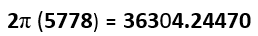The clear mirror feels it appropriate to show us that 36304.24470 = 27.52 x 48.00561… and that since the base measure of the encasing pyramid is 27.52 or 756.25 feet, its perimeter is 27.52 x 48 or 36300 inches, given that there are 4 sides and 12” to a foot. That is 4.24470 less than the Spherical Time circumference. It then reminds us that the Tower of Truth and encasing pyramid is (210 x 27.5) = 5775” tall, which is 3 less then 5778, and that 3 is derivative of the regression series of 27.5.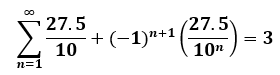It then brings up the Cube of 48 letters, the 12 YHVH (יהוה) that correspond to the 12 months of the lunar year and Cosmic Wheel. We are reminded that the cube root of 48, the edge length of the Cube of 48 Letters is 3.6342412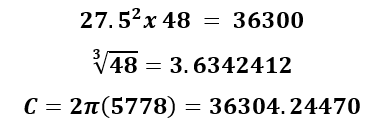Bringing up the Spiritual/Physical dichotomy in the Spherical Time equation the clear mirror shows us the two separate but equal spheres from the standpoint of the universe: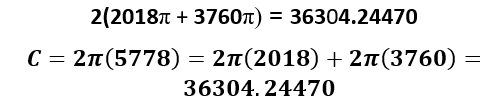It reminds us that within the resultant circumference is not only the sum of the first 1000 digits of Pi (π), 4470, but the relationship between the 44.70 hours of Physical Time in the sphere of 2018 that equal 24 hours of Spiritual Time in the sphere of 3760, and their relationship with the speed of light, 3760/2018 = 44.70/24 = 1.86282.

It is reminding us of this because the log of 5760, which is the year 2000 CE and the start of the 21st century equals 3.760422 and the First Holy Temple was destroyed in –422 CE and the Second Holy Temple was destroyed in 70 CE and the Hebrew word for seventy (70) has the gematria value of 422. It appears the 20 level Tower of Truth is also the Tower of Time.

It is also reminding us of this because the sum of the square roots (6.774) of the 10 cyclical remainders of the mod (27.5) application that equals the wavelength (6774 miles) of the Primal Frequency, less 10 times the square root of the 8th remainder in the cycle, or (6.77410.0909…) approximates 3.760.

It goes without saying that the only way this can work out this way is if the same Creator who designed the Pi (π) and Phi (φ) fields to the last digit; also designed the base 10 numbering system; the Hebrew Alef-bet; the various gematria cipher numbering systems of the Alef-bet; the dimensions and cosmic mechanisms of our universe; the speed of light; Planck’s Constant, and all the other constants for that matter; every aspect of the Torah; the basic history of mankind; the Wheels of the Cosmic Clock and time itself.

The question is not whether we are being shown this to get a few more people into Shul, or to awaken a critical mass before time runs out? Whether we are being shown this to stretch our minds or the boundaries of our consciousness? Whether this is just the blueprints to the real universe or a map to freedom and Heaven, the worlds beyond?

At one point in this journey, we were shown that there are exactly 58 or 390,625 words, letters, and verses in the Torah, and that much like the Fibonacci sequence, the quantity 390,625, each one representing a different letter, word, or verse in the Torah, began with 1 and then expanded because of 2.  The number One (1) divided by 2 = .5, then divided by 2 again and again 8 times gives us .00390625. This also works out to 1/256, the numerical value of the Ark of the Covenant. Viewing that repeated division not as an isolated series of mathematical operations but as a mathematic sequence or set (1, .5, .25, .125, ,0625, .03125, .015625, .0078125, .00390625…) and using Number Theory, or alternatively small gematria, this infinite set reduces to (1, 5, 7, 8, 4, 2, 1, 5, 7, 8, 4, 2…), an infinite repetitive pattern of 157842. Besides the obvious numeric allusions, we were shown that (1 + 5 + 7 + 8 + 4 + 2) = 27, as in the 27 positions of the Essential Cube of Creation and the corresponding 27 letters of the Alef-bet that the Torah is composed of. Moreover, the sum of the reduced divisional sequence through .00390625 is (1, 5, 7, 8, 13, 11, 19, 23, 25) = 112, as in the 112 Triplets of Creation.

The limit of these division is 2 as in the 2 Paths. If we were to add up all those divisions by 2, they would approach closer and closer to 2. Diluting the Light by half each iteration brings it closer to zero (0), but in aggregate it is 2, with 1 of that 2 being the original Source (1), and the rest diluted copies, fractal versions of the true Light.

We were shown that if the number doubles, which is the inverse of halves, the reverse sequence emerges (1, 2, 4, 8, 7, 5, 1, 2, 4, 8, 7, 5), and so the repeat sequence (1, 2, 4, 8, 7, 5) also equals 27.

This is what the YouTube videos like to tell people is “Telsa vortex math.” Tesla understood frequency, not gimmicks. Taking this resultant repetitive sequence backwards and forwards and subtracting the universe’s secret sequence (124875 + 157842 – 22727), we get 59990 = (60,00010), with Noach being the 10th in the lineage of Adam, Man.

Within the sequences 124875 and 157842 are the years 2487.5 that is 2488 HC and 5784 HC hidden between the 248 and 42 of the columns and rows of the Torah Matrix, the constant being 1, the Source.

Next, on this trip down memory lane are the 6 Essential Elements of Creation: the 4 sets of Triplets that total 16,965, along with the 4995 total from the Essential Cube of Creation, and the 48-Letter Cube Matrix with a value of (12 x 26) = 312. Altogether, the 6 Essential elements equal (16965 + 4995 + 312) = 22272, off from the secret sequence by exactly 455, the sum of the 3 Names Ehyeh (אהיה) associated with Binah and the Light of the Holy Temple.

For the first time we are noticing that all the associations with the secret key or coded sequence relate to 6, Zeir Anpin.  This continues with the 6 squared faces of the Essential Cube of Creation with their 12 diagonals that equal 22722, or (42 x 541), Israel.  While 22722 is (227275), as in the 5th Element of Creation, the Essential Cube, we realize that 22722/6 = 3787 and 22727/6 is 3787.8, relating both coded sequences to 378, the sum of the 27 positions/ordinal values in the Essential Cube of Creation, and to “He and His Name are One.”

The clear mirror further reminds us that the 7 dimensions (2148 + 2148 + 2256 + 2256 + 2328 + 2328 + 2442) of the same squared Essential Cube have an average (15906/7) of 2272.2.

The palms are eerily silent, the silver mercury bath dead still. It is so stone silent that we can hear our breathing and the pumping of our hearts.  The air does not stir; it does not even exist.

Then, after what feels like forever, the clear mirror shows us that the square root of the 3 main Torah elements, 625, the value of H’Keter, the crowning level, is equivalent to 22.727 cubits, 22.727 cycles of Hz.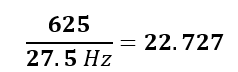The key to Creation was under our noses the entire time.  The mysterious secret sequence is the Frequency of Creation.

The clear mirror then squares this resultant frequency. It shows us 22.7272 = 516.516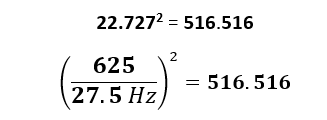This is the 516 times that Moses had to pray to bring about the final redemption.

We then realize that 516 is (2 x 258), as in the 258th Day in the Cosmic Wheel, the Day of Creation, the 25th of Elul, 6/25 on the Hebrew Calendar.

We also realize that 258 Days was the sum of the 42 days of the 42-Letter Name, and the 216 Days of the 72 Names, the 86 Triplets on Moses’ staff that was used to help manifest the splitting of the Sea. The Sea was split by binding it with Creation.

Those 516.516 hours are equivalent to 21.5215 days, as in the 6 Elemental Matrixes that form Zeir Anpin (42, 72, 27, 48, 11, 15) and equal 215. In the 21.5 hours from Rosh Hashanah though Hashanah Raba harmony can be achieved and the Torah reborn, the cycle refreshed on Simchat Torah.

The two prophetic phrases in the 42nd paragraph about the final redemption in the Song of the Sea both start off with 516 and both have a total gematria of 625 (keter) with the kolel.

The clear mirror has one more surprise for us, yet another mind-boggling revelation.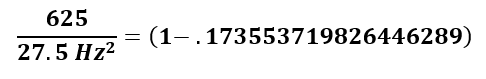We are speechless. The secret code of Creation directly relates to the mysterious 18-digit sequence built into our base 10-digit stream, the Fibonacci Numbers, the Tower of Truth, and who knows where else it will turn up.  And they all relate through the Torah (58), the Primal Frequency of the universe (27.5 Hz) and Phi (φ).

### Numeric Tetrahedra

Across the now pale blue sky, we see the set of all the positive integers, and one by one they start flying away, then by the tens, hundreds, thousands, millions, and billions. We are left with only 6 numbers: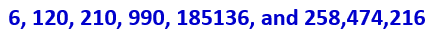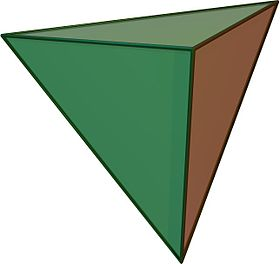The clear mirror explains that there are only 6 Triangular Numbers that are the product of 3 consecutive integers.  We are told to think of them as numeric tetrahedra with the 3 consecutive integers on 3 vertices and their product on the 4th.  The first one, 6, is the product of (1 x 2 x 3). It is akin to the Tetrahedron of the 1st Triplet (אבג) of the 42-Letter Name Matrix of numerical values (1 x 2 x 3).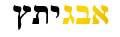The next of the 6 is 120, comprised of the product of (4 x 5 x 6) corresponding to the consecutive (דהו) in the middle of Yehuda (יהודה) found 42 times in the Torah.  This is the 15th Triangular Number as in the (יה) surrounding the triplet (דהו) in Yehuda (יהודה).

The 3rd of 6 is the ubiquitous 210, at the core of the Cosmic structure of the 6 dimensions of Zeir Anpin and physicality. It is the product of (5 x 6 x 7) so naturally 5 x 42.

We then see that (6 + 120) = 126, the value of each of the 6 faces of the Essential Cube of Creation, and 3 x 42.  Collectively they are the 3rd and 15th Triangular Numbers as in (3 + 15) = 18.

And then cumulatively, that sum (126 + 210) = 336, as in the 336 Letters in the 112 Triplets of Creation, and 8 x 42.  As we know so well 210 is the 20th Triangular Number, and that (120 + 210) = 330, the base measure, the harmonic (12 x 27.5) of the encasing pyramid to the 210 level Tower of Truth.

The 4th Tetrahedral number is 990 or (9 x 110), the convergence point, and thus 36 intervals of 27.5 Hz. It is the 44th Triangular Number.

The 5th Tetrahedral number jumps all the way to the 608th Triangular Number, the product of (56 x 57 x 58). It is (186,282 – 185,136) = 1146 less than the speed-of-light. Whether this 1146 relates to the 1143 from the 72 Triplet Matrix or to the 11466 square miles of Israel we can only speculate. Nonetheless (185,136 + 990 + 210) is exactly 54 more than the speed-of-light and (185,136 + 990 + 210 + 120 + 6) is 180 more than the speed-of-light. We can only assume that everything has its place and purpose.

The final and 6th of these special Tetrahedral numbers is 258,474,216. We immediately recognize the 258th Day, the Day of Creation from the Cosmic Wheel, followed by 474, Da’at, and the 216 Letters in the 72 Triplets of Creation in the Cosmic Wheel.

We also notice for the first time that the palindromic Da’at, the realm of Moshiach, 474, less the palindromic 363 Days in the Cosmic Wheel and Spherical Time and H’Moshiach is the palindromic 111, the Primordial Alef (אלף) and 1st Triplet from the Essential Cube of Creation.

The clear mirrored pool lets us know that 258,474,216 is the product of (636 x 637 x 638).

It then tells us that the 9-digit special Tetrahedral number is the 22736th Triangular Number or (2273622727) = 9 more than the secret code to the Cosmos.

The still small voice echoes from far away, “Read the map!”

Shabbat Shalom,

Ezra